# HITRAN spectra¶

The absorption coefficient of all HITRAN species (see `MOLECULES_LIST_EQUILIBRIUM`) is calculated in plot_all_hitran_spectra.py at 300 K, 1 atm for the first isotope:

• 1 `'H2O'` : Water (spectrum)

• 2 `'CO2'` : Carbon Dioxide (spectrum)

• 3 `'O3'` : Ozone (spectrum)

• 4 `'N2O'` : Nitrogen oxide (spectrum)

• 5 `'CO'` : Carbon Monoxide (spectrum)

• 6 `'CH4'` : Methane (spectrum)

• 7 `'O2'` : Oxygen

• 8 `'NO'` : Nitric Oxide (spectrum)

• 9 `'SO2'` : Sulfur Dioxide (spectrum)

• 10 `'NO2'` : Nitrogen Dioxide (spectrum)

• 11 `'NH3'` : Ammonia (spectrum)

• 12 `'HNO3'` : Nitric Acid (spectrum)

• 13 `'OH'` : Hydroxyl (spectrum)

• 14 `'HF'` : Hydrogen Fluoride (spectrum)

• 15 `'HCl'` : Hydrogen Chloride (spectrum)

• 16 `'HBr'` : Hydrogen Bromide (spectrum)

• 17 `'HI'` : Hydrogen Iodide (spectrum)

• 18 `'ClO'` : Chlorine Monoxide (spectrum)

• 19 `'OCS'` : Carbonyl Sulfide (spectrum)

• 20 `'H2CO'` : Formaldehyde (spectrum)

• 21 `'HOCl'` : Hypochlorous Acid (spectrum)

• 22 `'N2'` : Nitrogen

• 23 `'HCN'` : Hydrogen Cyanide

• 24 `'CH3Cl'` : Methyl Chloride (spectrum)

• 25 `'H2O2'` : Hydrogen Peroxide (spectrum)

• 26 `'C2H2'` : Acetylene (spectrum)

• 27 `'C2H6'` : Ethane (spectrum)

• 28 `'PH3'` : Phosphine (spectrum)

• 29 `'COF2'` : Carbonyl Fluoride (spectrum)

• 30 `'SF6'` : Sulfur Hexafluoride

• 31 `'H2S'` : Hydrogen Sulfide (spectrum)

• 32 `'HCOOH'` : Formic Acid (spectrum)

• 33 `'HO2'` : Hydroperoxyl (spectrum)

• 34 `'O'` : Oxygen Atom

• 35 `'ClONO2'` : Chlorine Nitrate

• 36 `'NO+'` : Nitric Oxide Cation (spectrum)

• 37 `'HOBr'` : Hypobromous Acid

• 38 `'C2H4'` : Ethylene

• 39 `'CH3OH'` : Methanol

• 40 `'CH3Br'` : Methyl Bromide

• 41 `'CH3CN'` : Acetonitrile

• 42 `'CF4'` : CFC-14

• 43 `'C4H2'` : Diacetylene

• 44 `'HC3N'` : Cyanoacetylene

• 45 `'H2'` : Hydrogen

• 46 `'CS'` : Carbon Monosulfide

• 47 `'SO3'` : Sulfur trioxide

• 48 `'C2N2'` : Cyanogen

• 49 `'COCl2'` : Phosgene

The code to calculate each molecule is shown below:

## 1. H2O¶

• 1 `'H2O'` : Water absorption coefficient (opacity) at 300 K

```s = calc_spectrum(wavelength_min=1000,
wavelength_max=20000,
Tgas=300,
pressure=1,
molecule='H2O',
optimization=None,
cutoff=1e-23,
isotope='1')
s.plot('abscoeff', wunit='nm')
```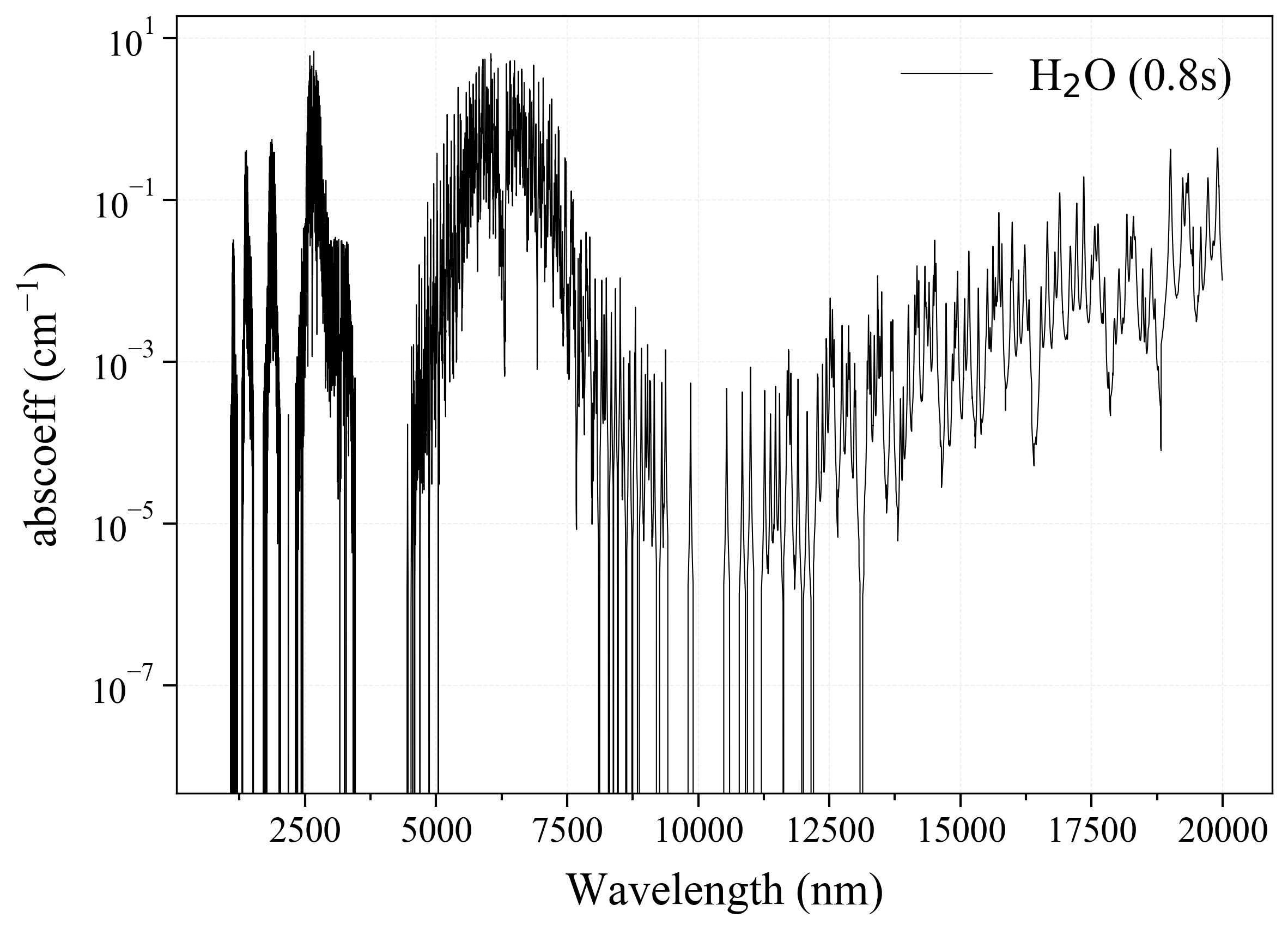## 2. CO2¶

• 2 `'CO2'` : Carbon Dioxide absorption coefficient (opacity) at 300 K

```s = calc_spectrum(wavelength_min=1000,
wavelength_max=20000,
Tgas=300,
pressure=1,
molecule='CO2',
optimization=None,
cutoff=1e-23,
isotope='1')
s.plot('abscoeff', wunit='nm')
```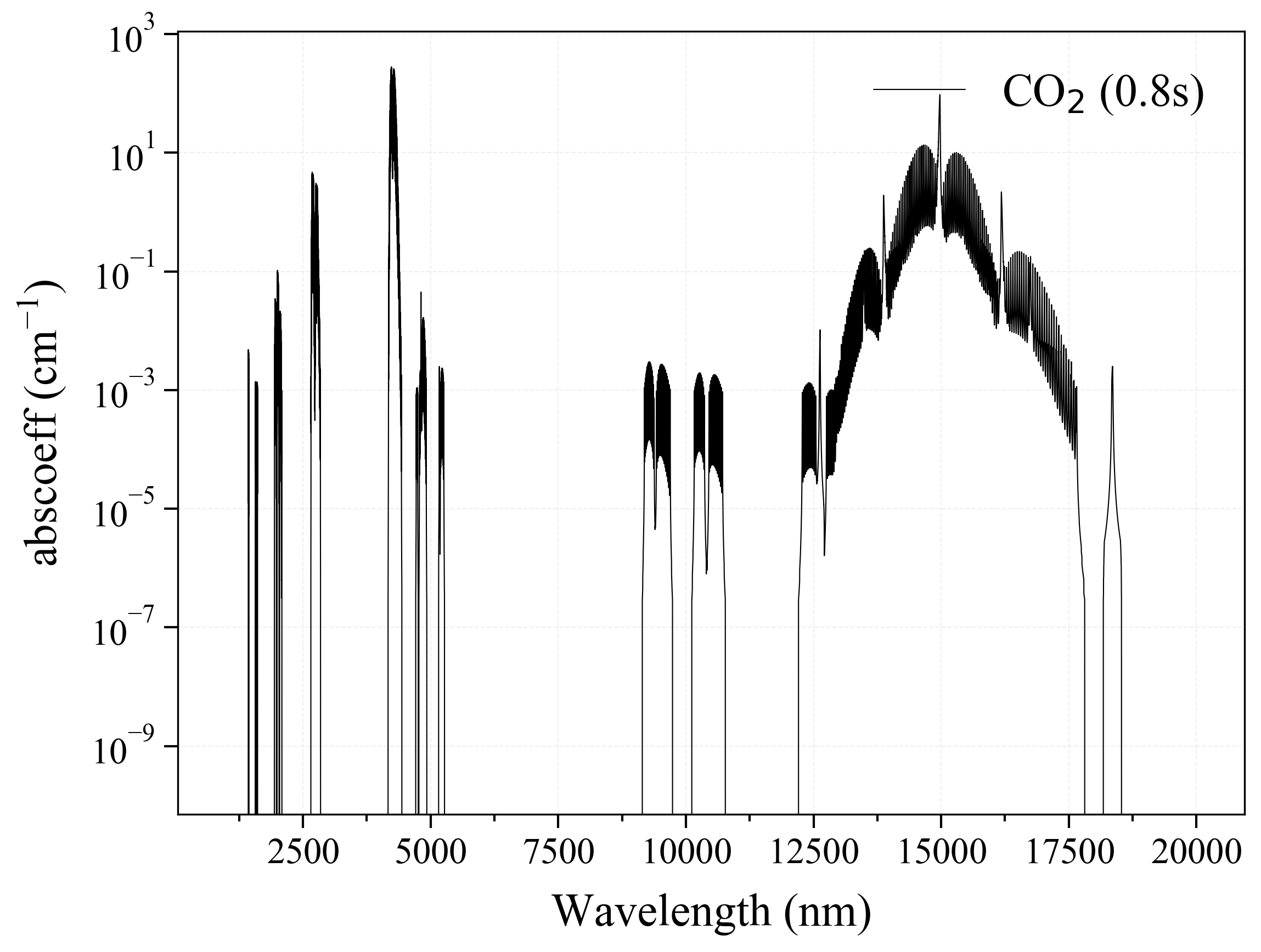3. O3 =====-

• 3 `'O3'` : Ozone absorption coefficient (opacity) at 300 K

```s = calc_spectrum(wavelength_min=1000,
wavelength_max=20000,
Tgas=300,
pressure=1,
molecule='O3',
optimization=None,
cutoff=1e-23,
isotope='1')
s.plot('abscoeff', wunit='nm')
```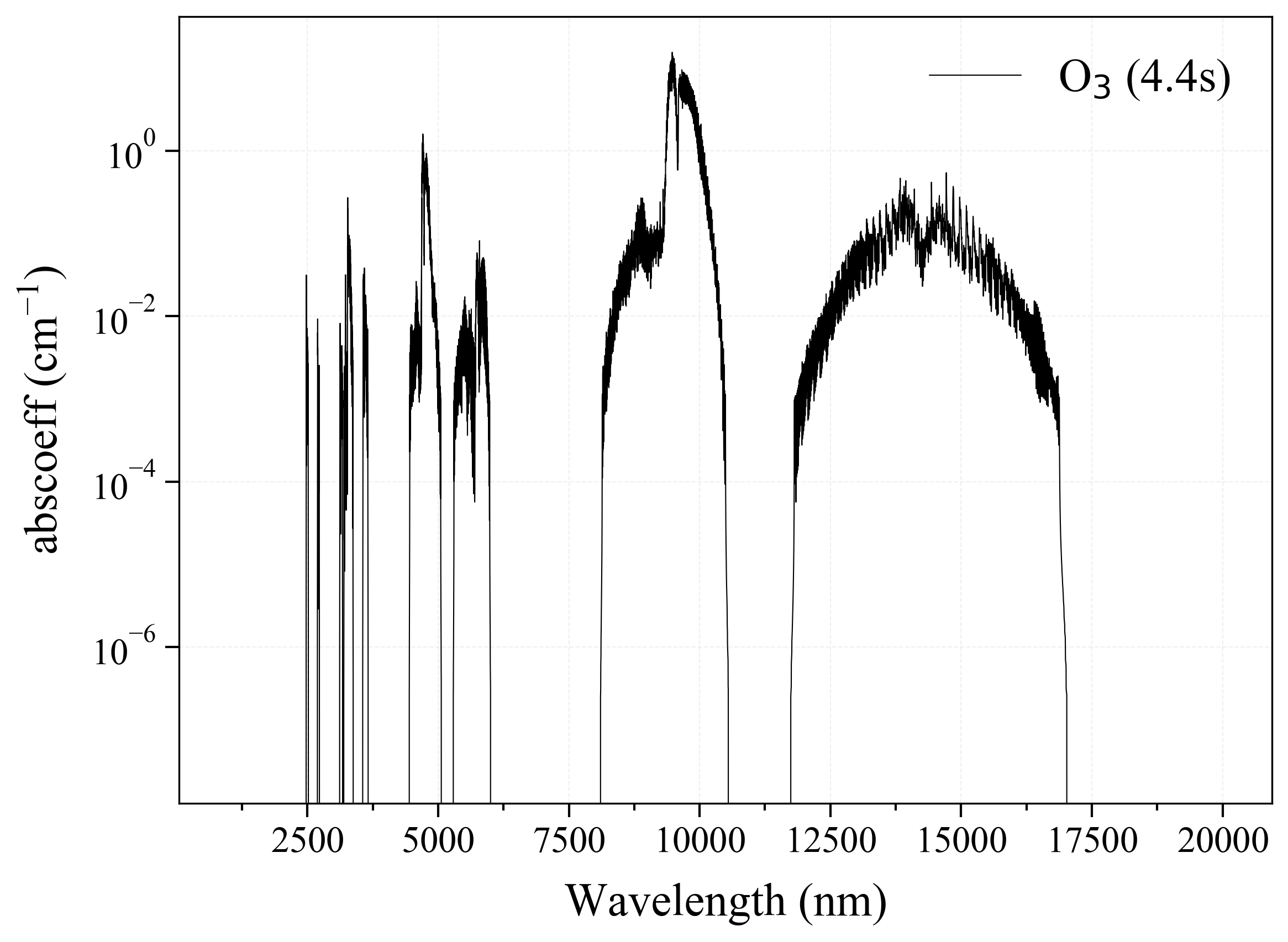## 4. N2O¶

• 4 `'N2O'` : Nitrogen oxide absorption coefficient (opacity) at 300 K

```s = calc_spectrum(wavelength_min=1000,
wavelength_max=20000,
Tgas=300,
pressure=1,
molecule='N2O',
optimization=None,
cutoff=1e-23,
isotope='1')
s.plot('abscoeff', wunit='nm')
```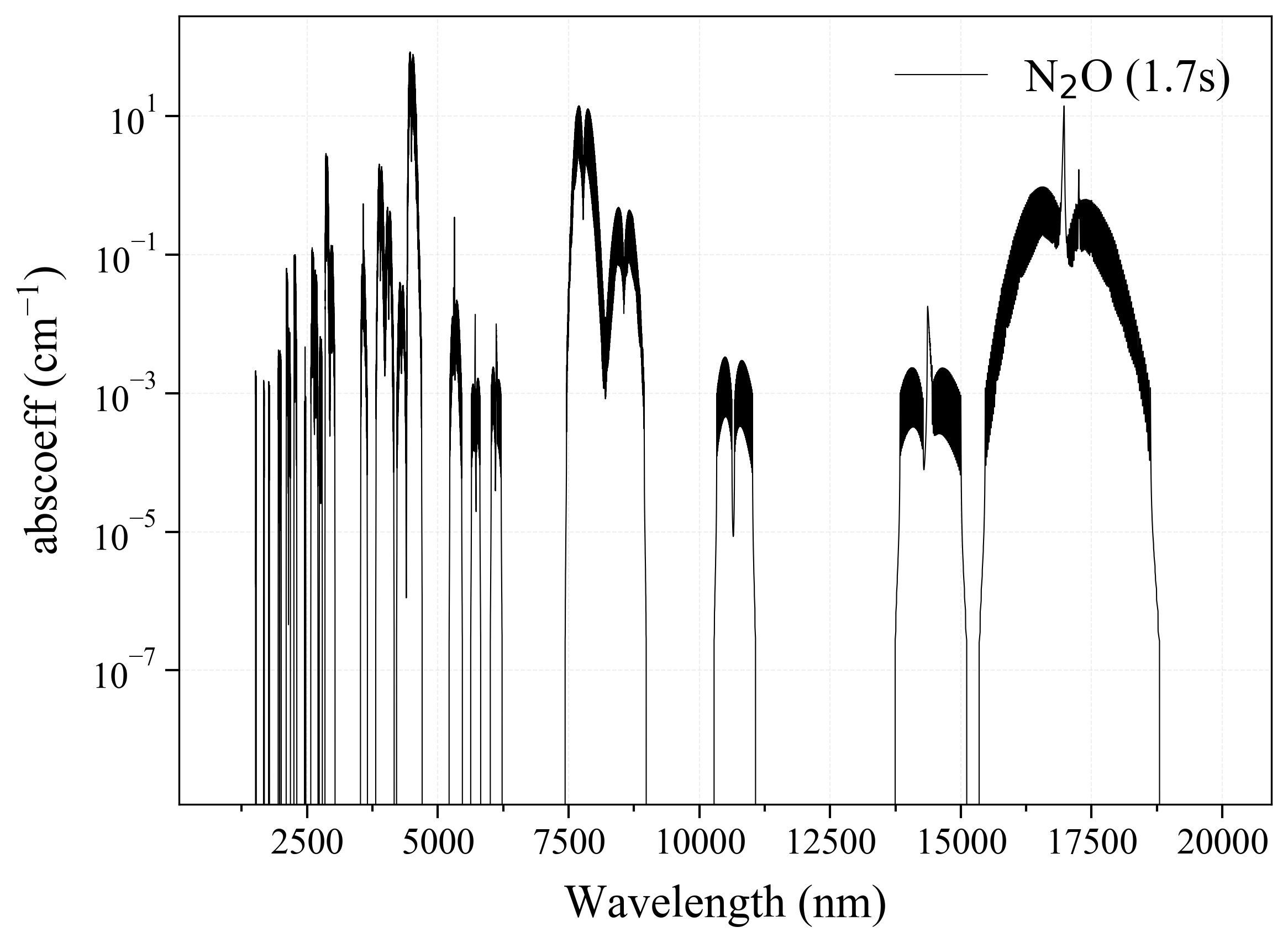## 5. CO¶

• 5 `'CO'` : Carbon Monoxide absorption coefficient (opacity) at 300 K

```s = calc_spectrum(wavelength_min=1000,
wavelength_max=20000,
Tgas=300,
pressure=1,
molecule='CO',
optimization=None,
cutoff=1e-23,
isotope='1')
s.plot('abscoeff', wunit='nm')
```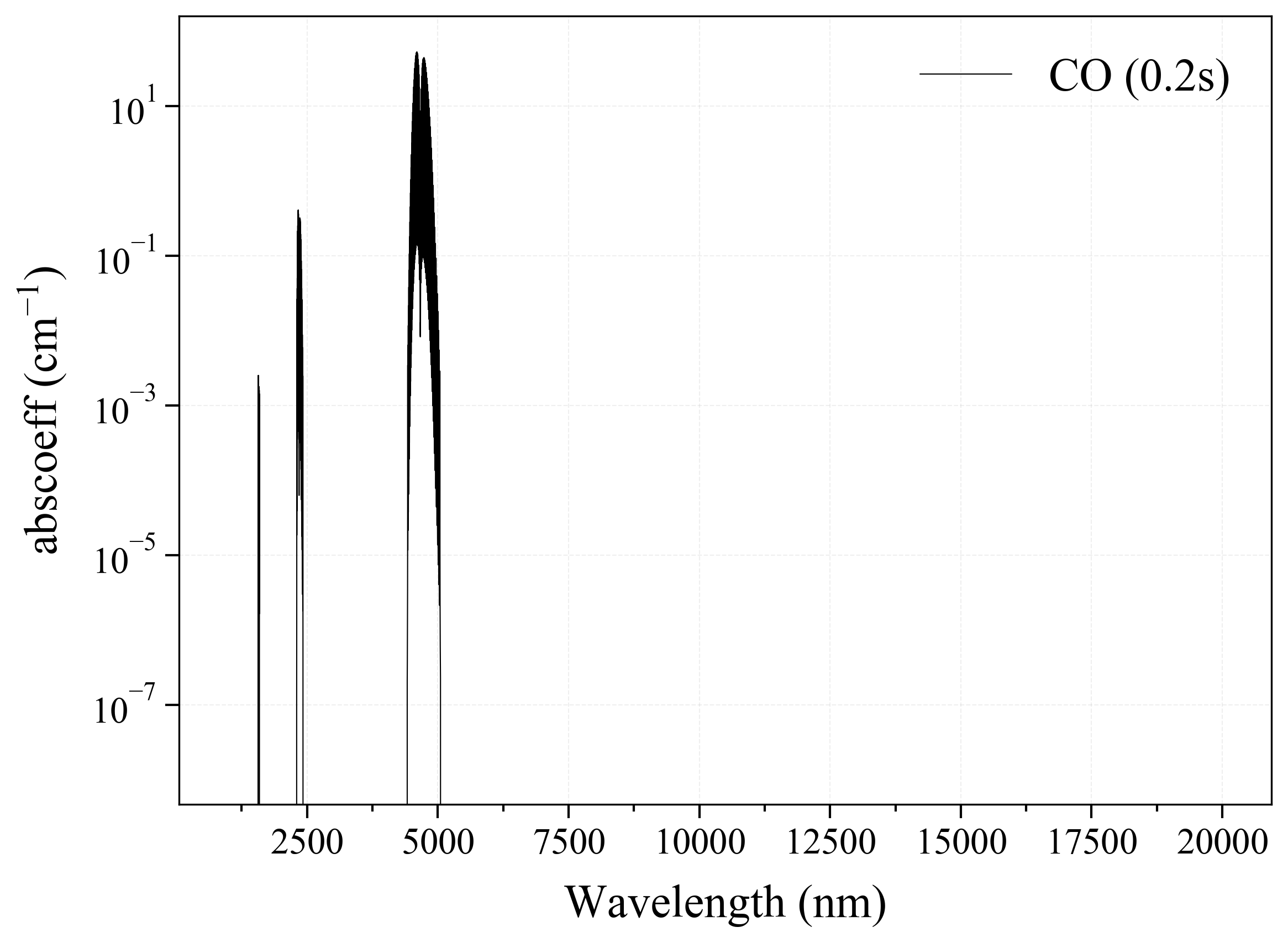## 6. CH4¶

• 6 `'CH4'` : Methane absorption coefficient (opacity) at 300 K

```s = calc_spectrum(wavelength_min=1000,
wavelength_max=20000,
Tgas=300,
pressure=1,
molecule='CH4',
optimization=None,
cutoff=1e-23,
isotope='1')
s.plot('abscoeff', wunit='nm')
```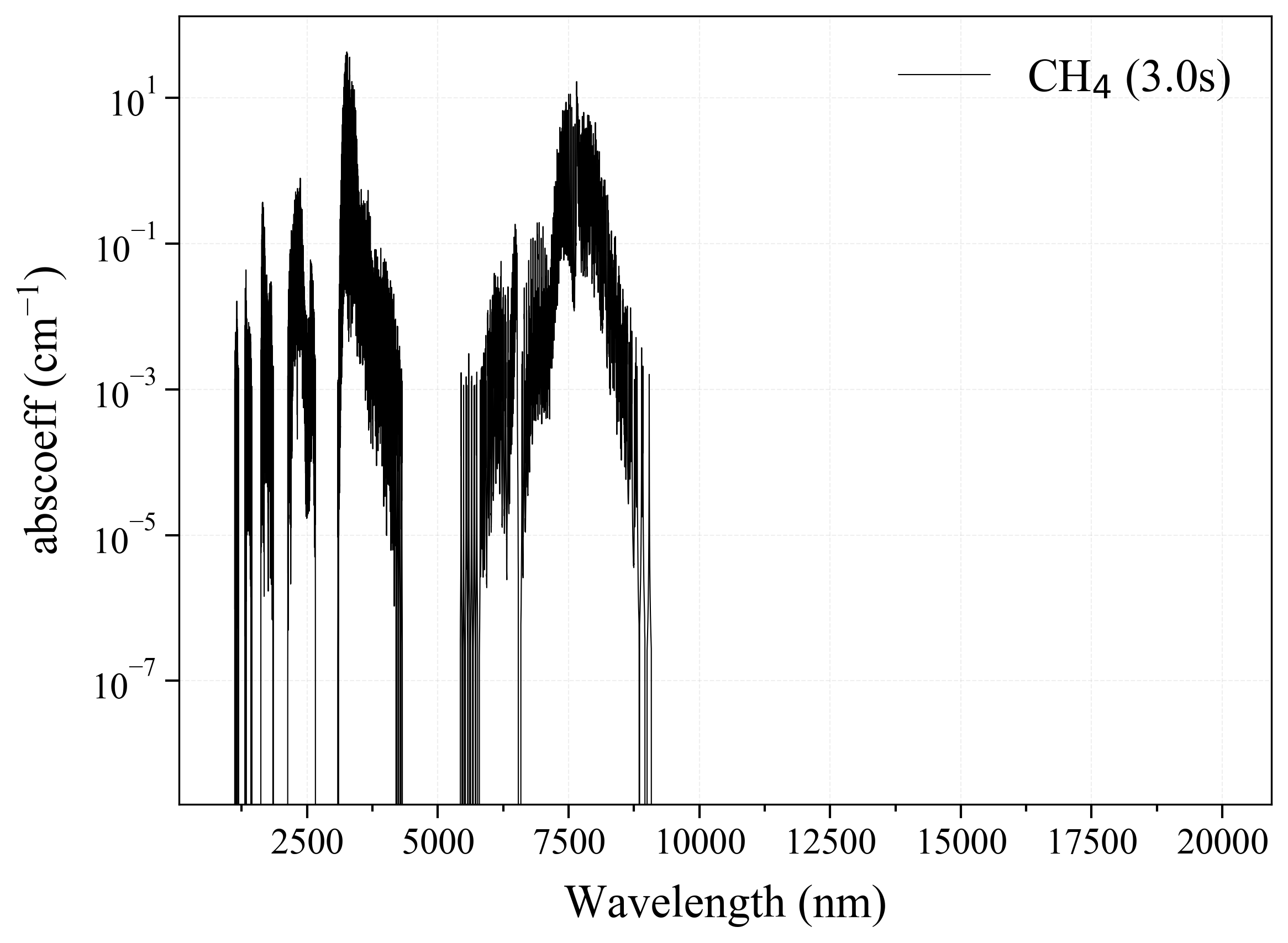7. O2 =====-

• 7 `'O2'` : Oxygen absorption coefficient (opacity) at 300 K : no lines for `isotope='1'` (symmetric!)

## 8. NO¶

• 8 `'NO'` : Nitric Oxide absorption coefficient (opacity) at 300 K

```s = calc_spectrum(wavelength_min=1000,
wavelength_max=20000,
Tgas=300,
pressure=1,
molecule='NO',
optimization=None,
cutoff=1e-23,
isotope='1')
s.plot('abscoeff', wunit='nm')
```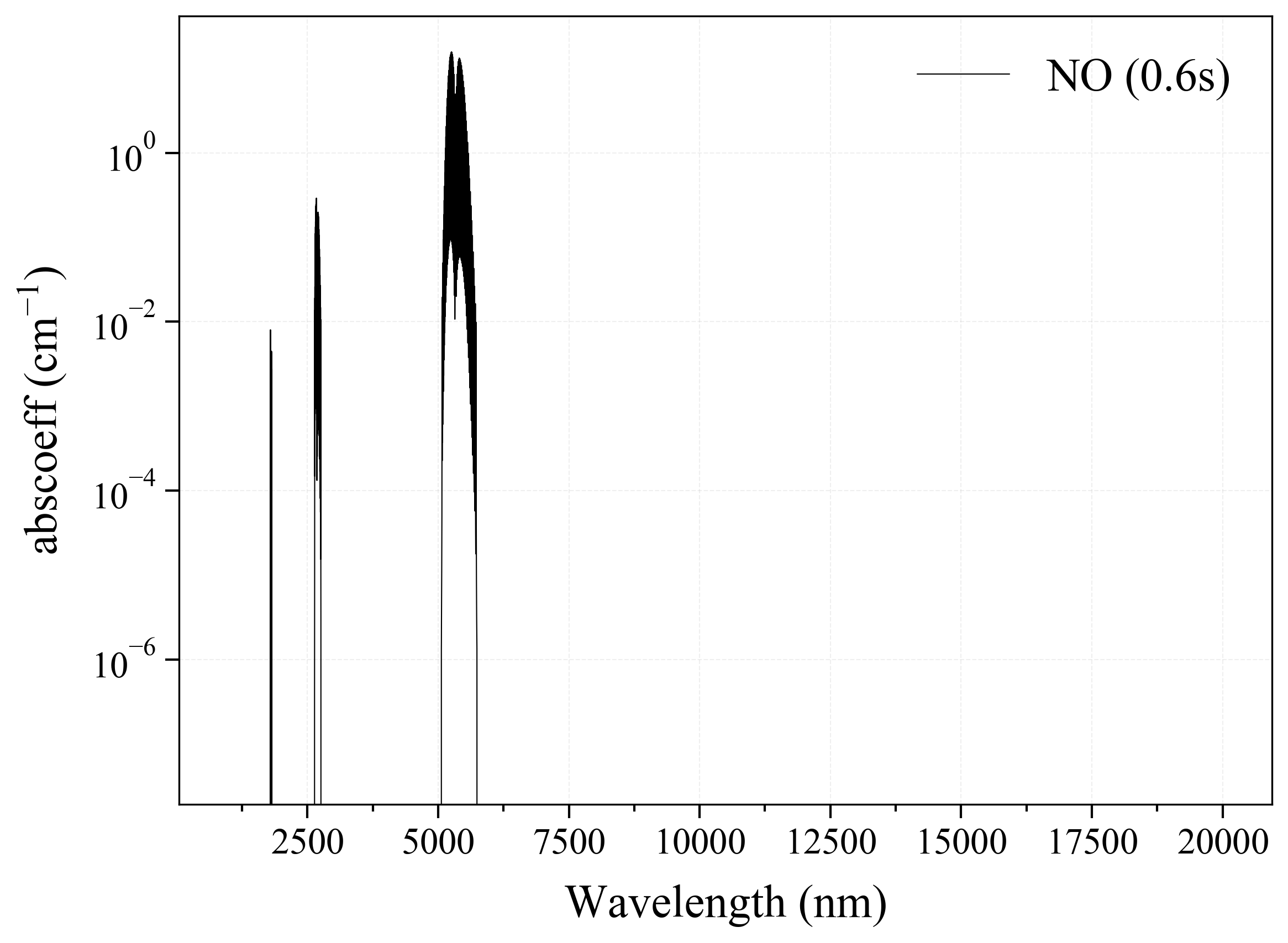## 9. SO2¶

• 9 `'SO2'` : Sulfur Dioxide absorption coefficient (opacity) at 300 K

```s = calc_spectrum(wavelength_min=1000,
wavelength_max=20000,
Tgas=300,
pressure=1,
molecule='SO2',
optimization=None,
cutoff=1e-23,
isotope='1')
s.plot('abscoeff', wunit='nm')
```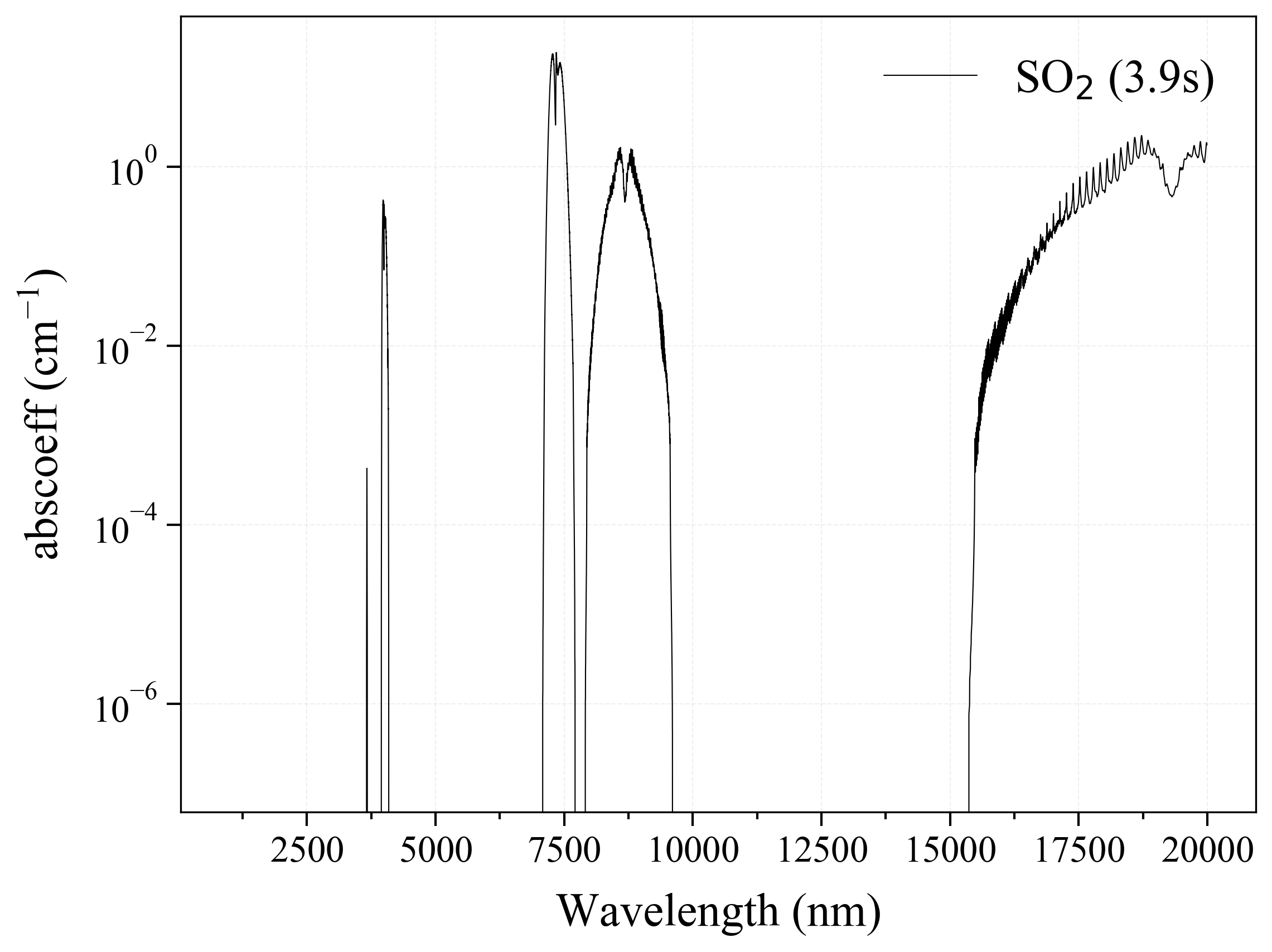## 10. NO2¶

• 10 `'NO2'` : Nitrogen Dioxide absorption coefficient (opacity) at 300 K

```s = calc_spectrum(wavelength_min=1000,
wavelength_max=20000,
Tgas=300,
pressure=1,
molecule='NO2',
optimization=None,
cutoff=1e-23,
isotope='1')
s.plot('abscoeff', wunit='nm')
```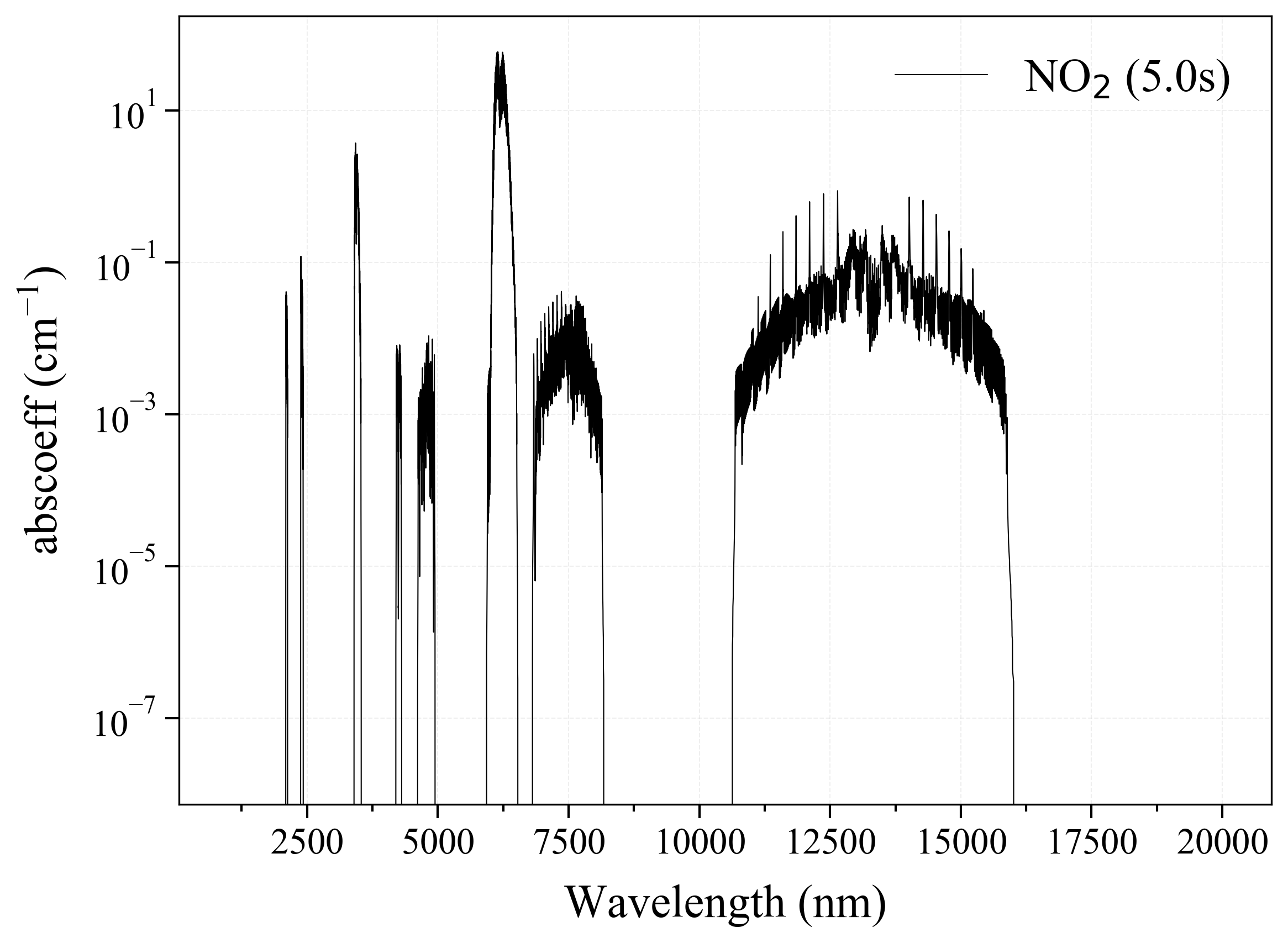## 11. NH3¶

• 11 `'NH3'` : Ammonia absorption coefficient (opacity) at 300 K

```s = calc_spectrum(wavelength_min=1000,
wavelength_max=20000,
Tgas=300,
pressure=1,
molecule='NH3',
optimization=None,
cutoff=1e-23,
isotope='1')
s.plot('abscoeff', wunit='nm')
```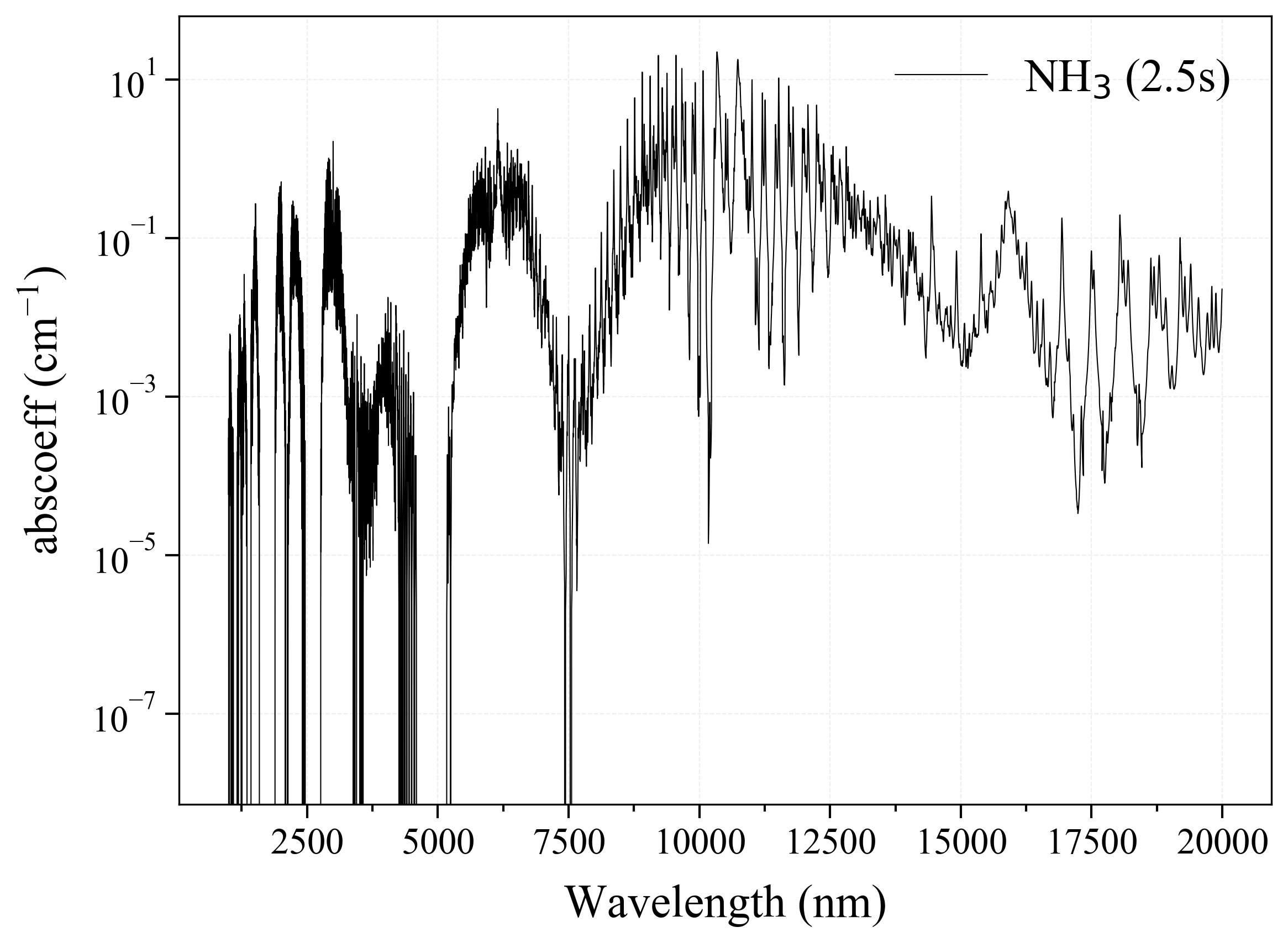## 12. HNO3¶

• 12 `'HNO3'` : Nitric Acid absorption coefficient (opacity) at 300 K

```s = calc_spectrum(wavelength_min=1000,
wavelength_max=20000,
Tgas=300,
pressure=1,
molecule='HNO3',
optimization=None,
cutoff=1e-23,
isotope='1')
s.plot('abscoeff', wunit='nm')
```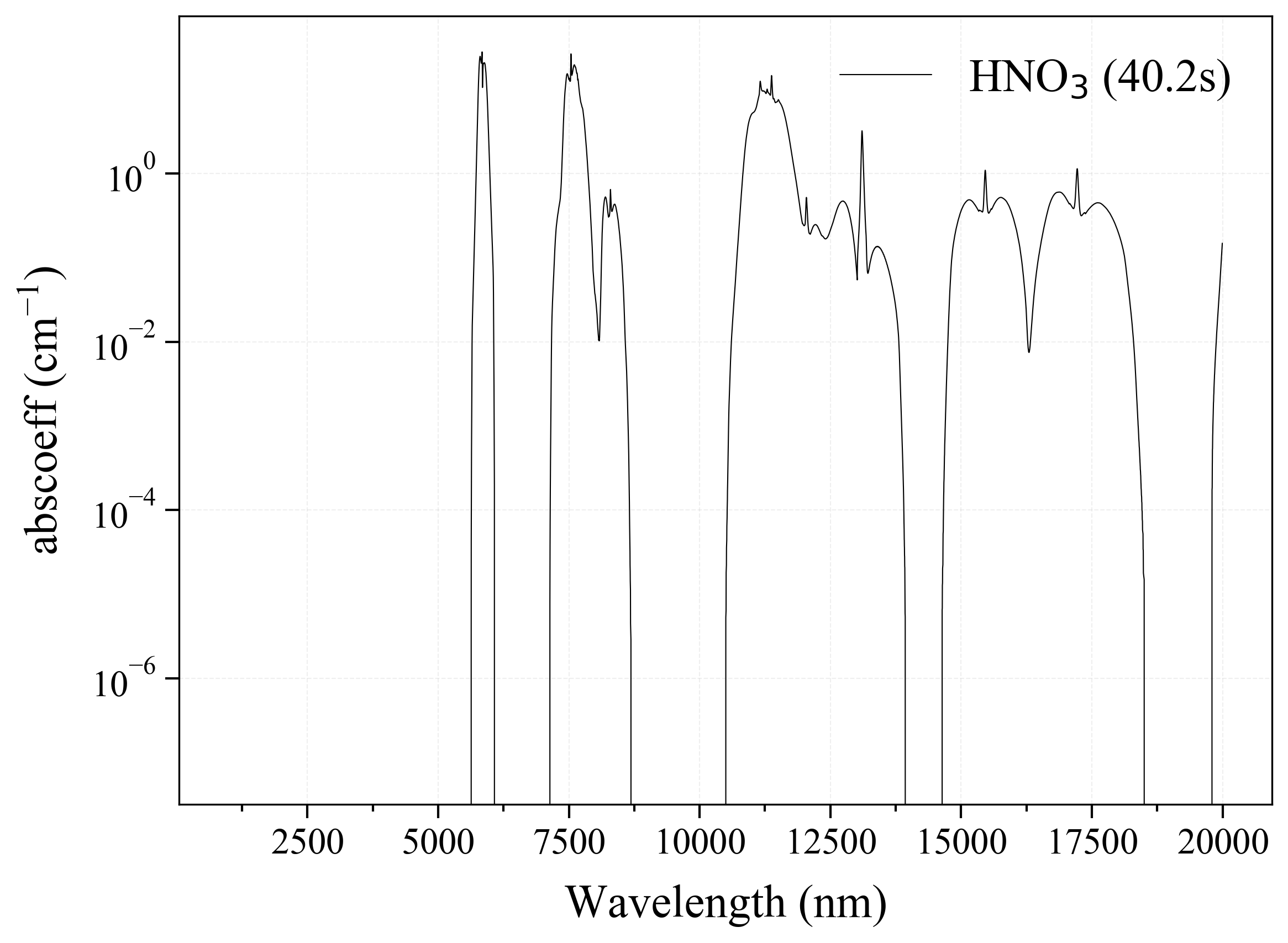## 13. OH¶

• 13 `'OH'` : Hydroxyl absorption coefficient (opacity) at 300 K

```s = calc_spectrum(wavelength_min=1000,
wavelength_max=20000,
Tgas=300,
pressure=1,
molecule='OH',
optimization=None,
cutoff=1e-23,
isotope='1')
s.plot('abscoeff', wunit='nm')
```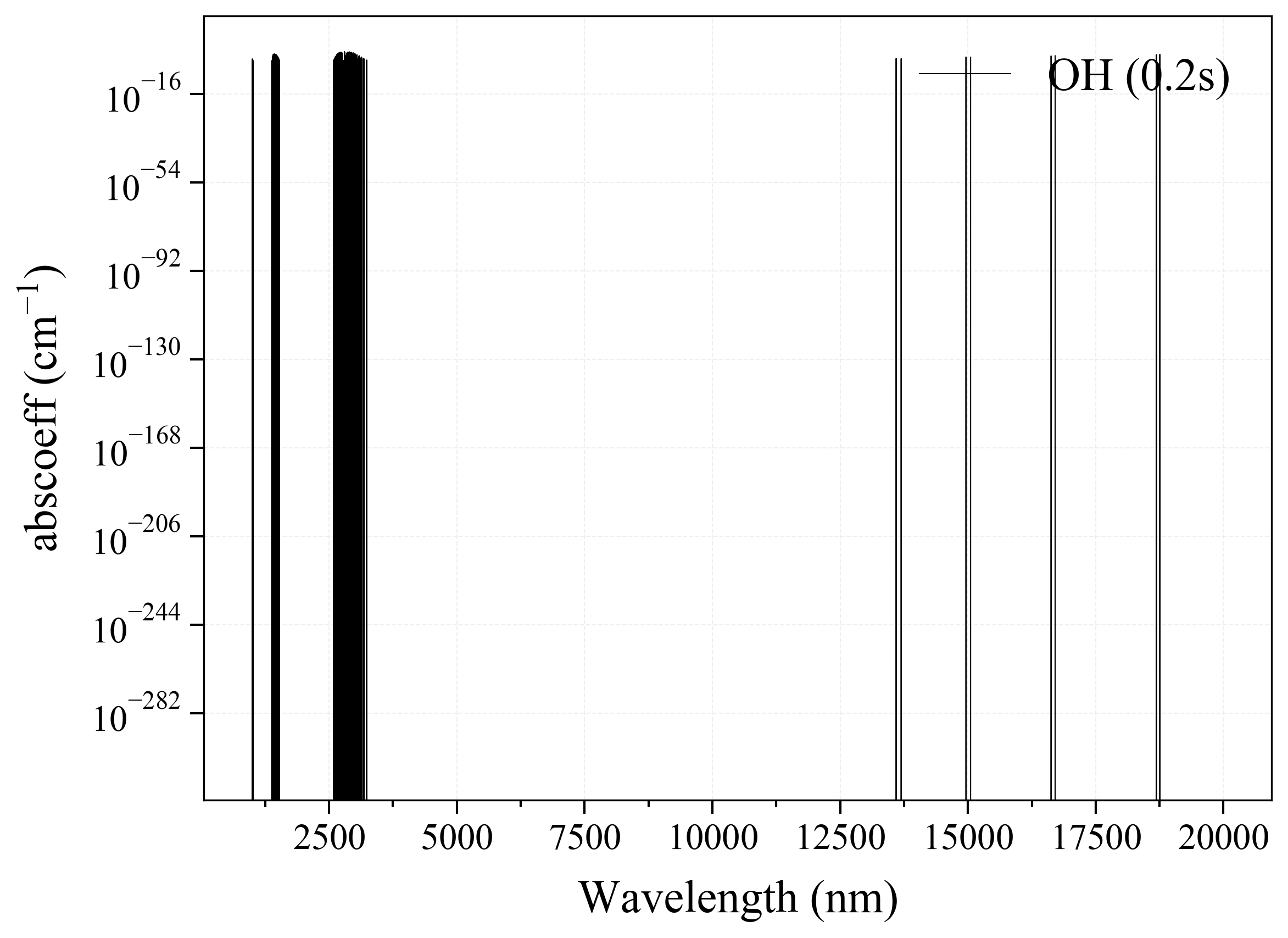## 14. HF¶

• 14 `'HF'` : Hydrogen Fluoride absorption coefficient (opacity) at 300 K

```s = calc_spectrum(wavelength_min=1000,
wavelength_max=20000,
Tgas=300,
pressure=1,
molecule='HF',
optimization=None,
cutoff=1e-23,
isotope='1')
s.plot('abscoeff', wunit='nm')
```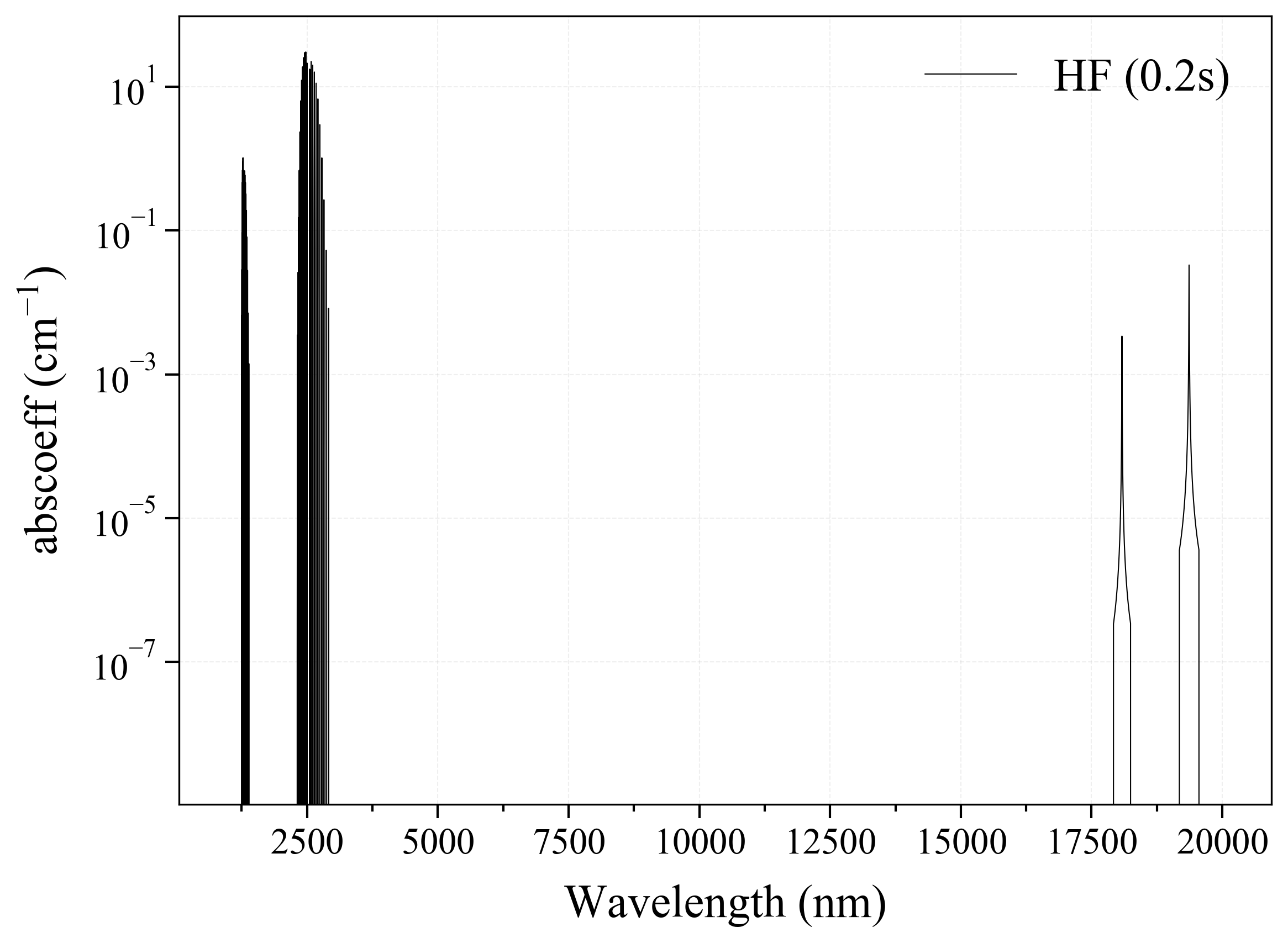## 15. HCl¶

• 15 `'HCl'` : Hydrogen Chloride absorption coefficient (opacity) at 300 K

```s = calc_spectrum(wavelength_min=1000,
wavelength_max=20000,
Tgas=300,
pressure=1,
molecule='HCl',
optimization=None,
cutoff=1e-23,
isotope='1')
s.plot('abscoeff', wunit='nm')
```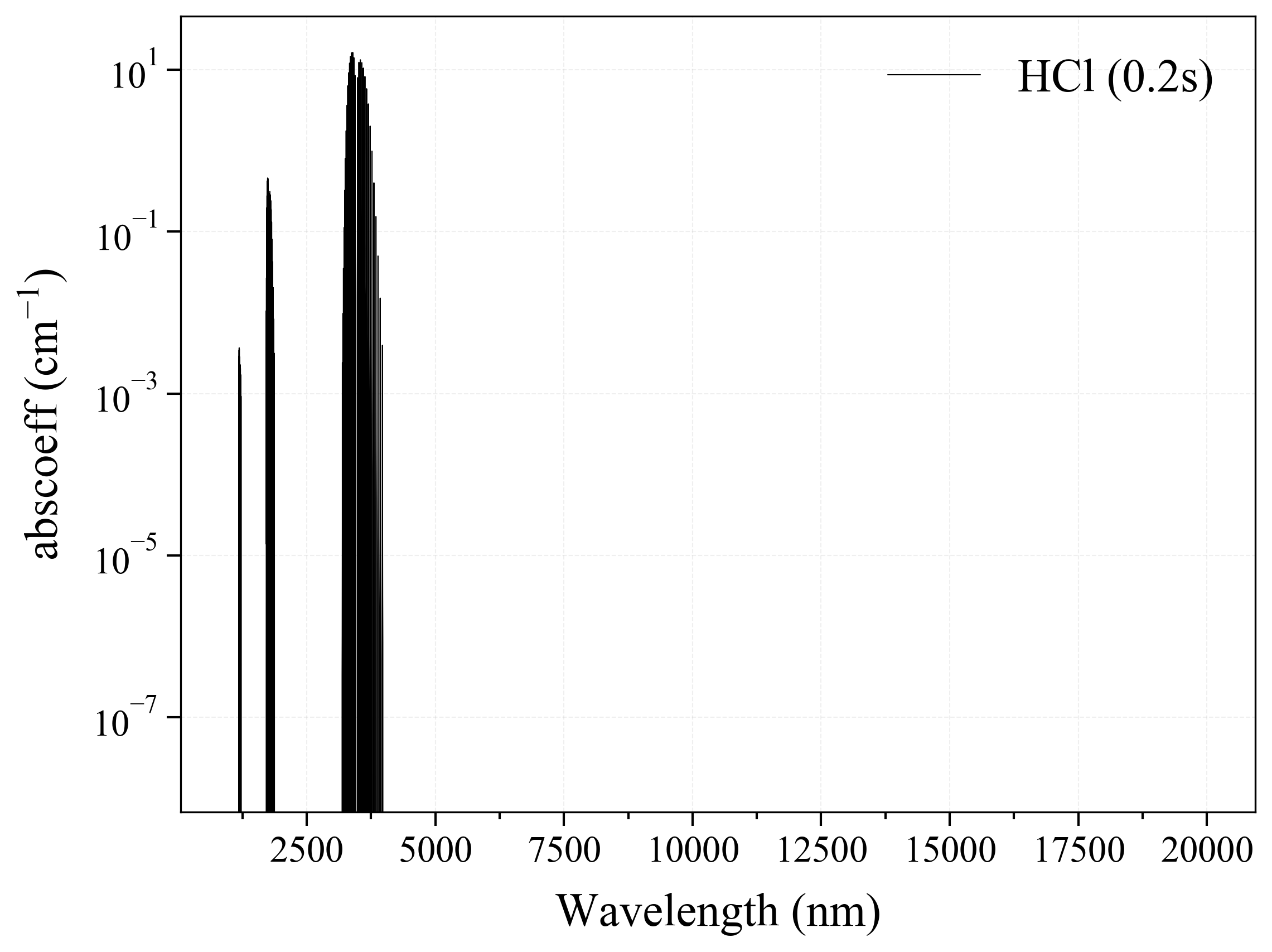## 16. HBr¶

• 16 `'HBr'` : Hydrogen Bromide absorption coefficient (opacity) at 300 K

```s = calc_spectrum(wavelength_min=1000,
wavelength_max=20000,
Tgas=300,
pressure=1,
molecule='HBr',
optimization=None,
cutoff=1e-23,
isotope='1')
s.plot('abscoeff', wunit='nm')
```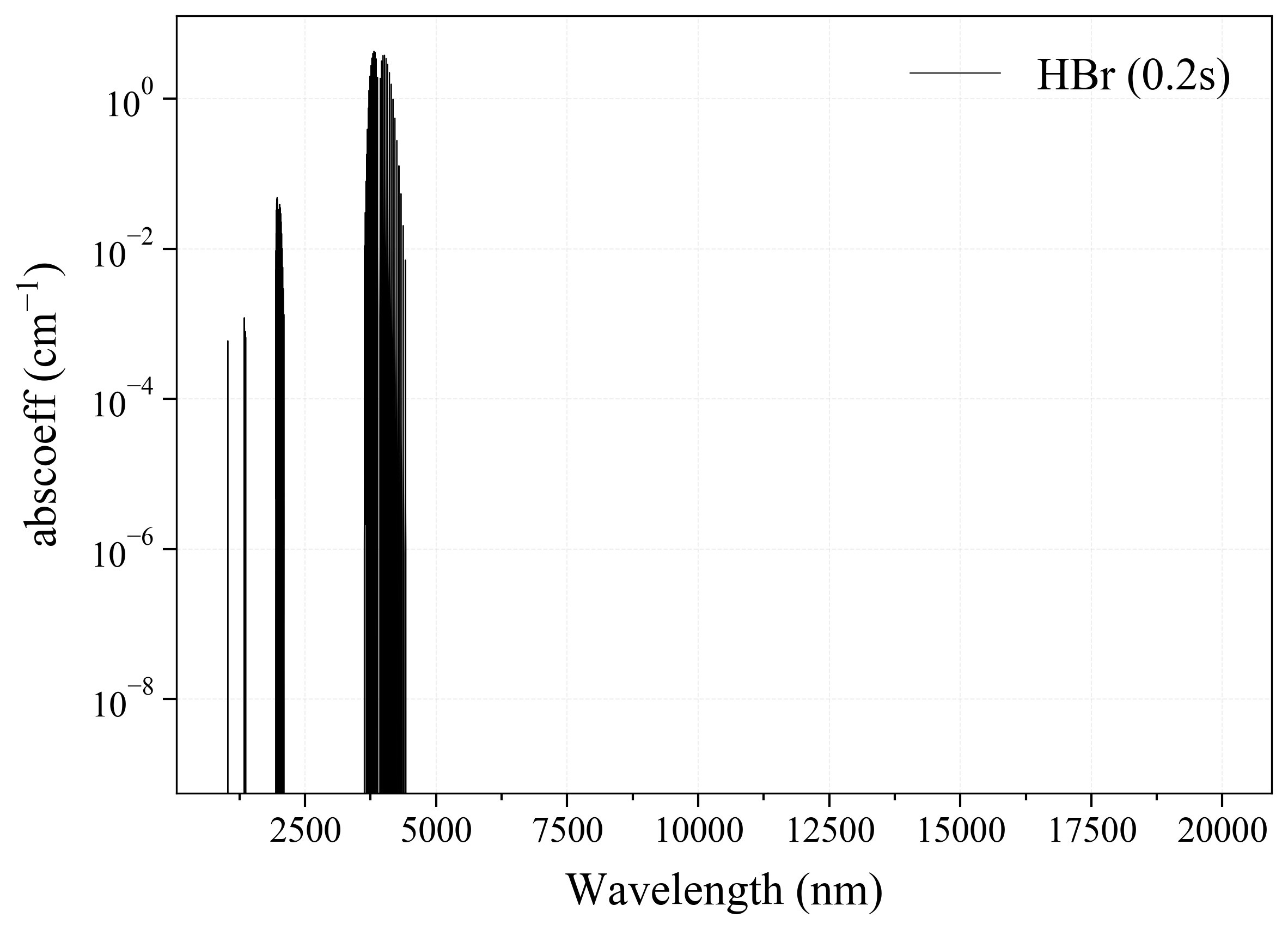## 17. HI¶

• 17 `'HI'` : Hydrogen Iodide absorption coefficient (opacity) at 300 K

```s = calc_spectrum(wavelength_min=1000,
wavelength_max=20000,
Tgas=300,
pressure=1,
molecule='HI',
optimization=None,
cutoff=1e-23,
isotope='1')
s.plot('abscoeff', wunit='nm')
```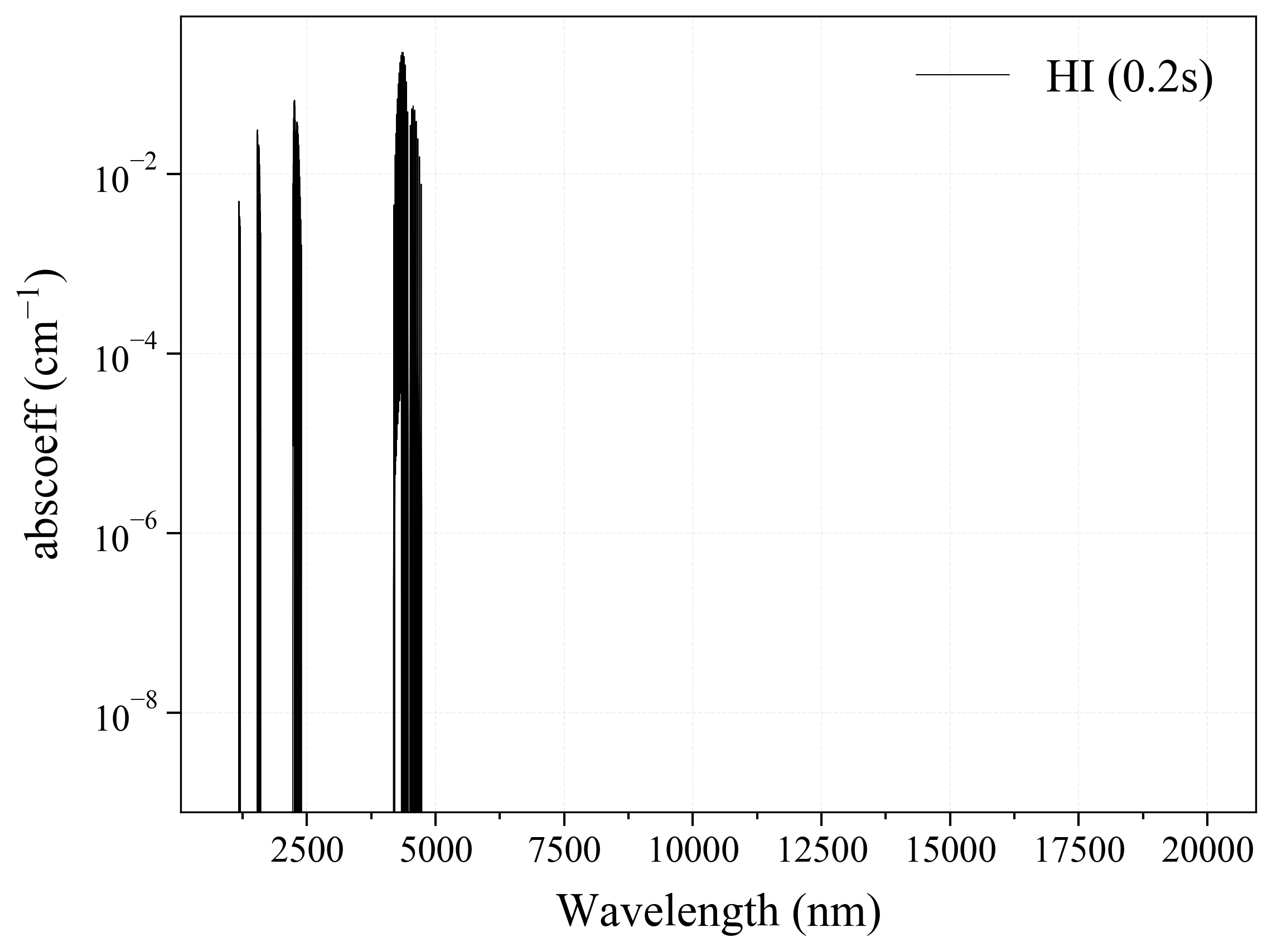## 18. ClO¶

• 18 `'ClO'` : Chlorine Monoxide absorption coefficient (opacity) at 300 K

```s = calc_spectrum(wavelength_min=1000,
wavelength_max=20000,
Tgas=300,
pressure=1,
molecule='ClO',
optimization=None,
cutoff=1e-23,
isotope='1')
s.plot('abscoeff', wunit='nm')
```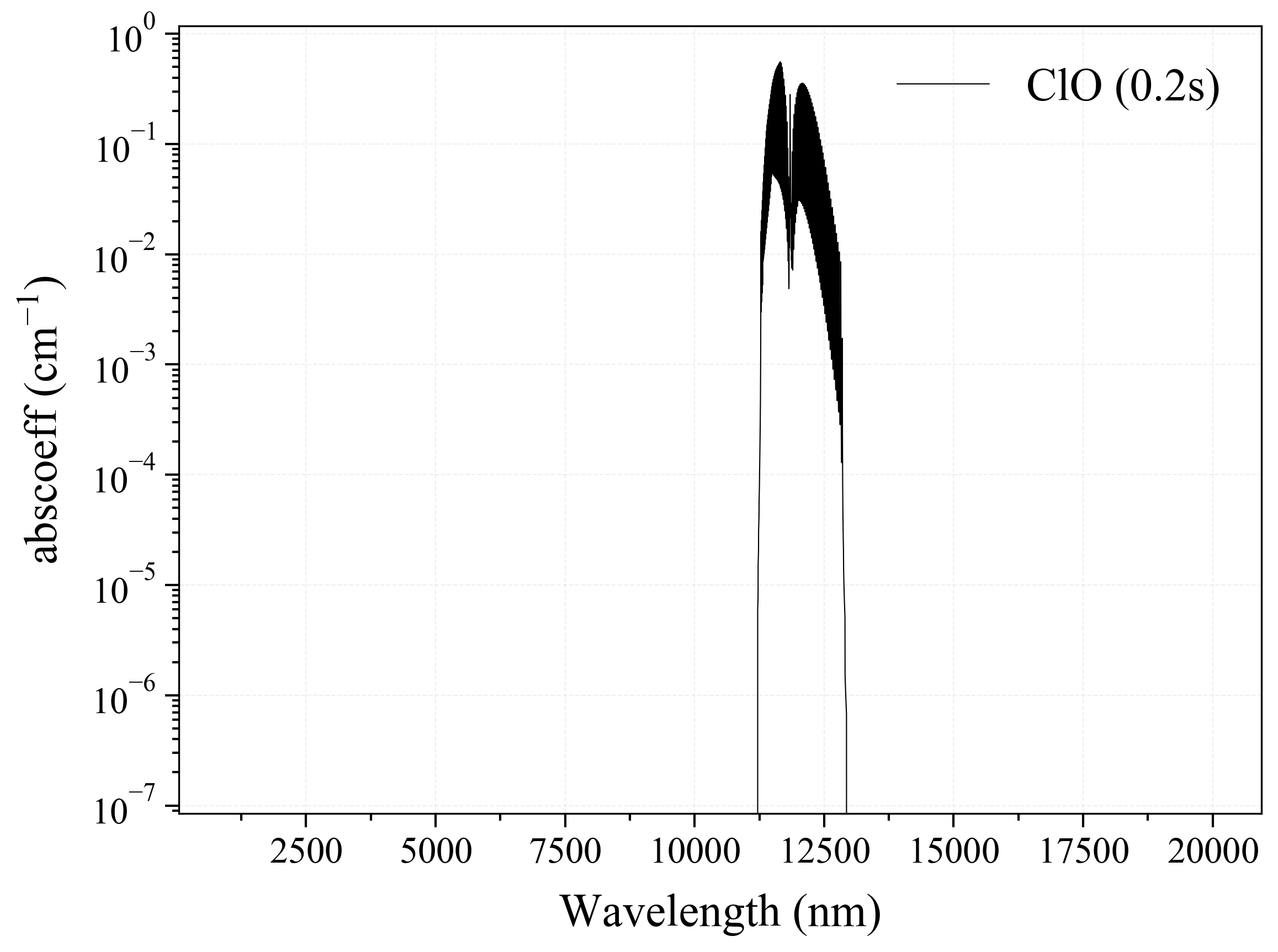## 19. OCS¶

• 19 `'OCS'` : Carbonyl Sulfide absorption coefficient (opacity) at 300 K

```s = calc_spectrum(wavelength_min=1000,
wavelength_max=20000,
Tgas=300,
pressure=1,
molecule='OCS',
optimization=None,
cutoff=1e-23,
isotope='1')
s.plot('abscoeff', wunit='nm')
```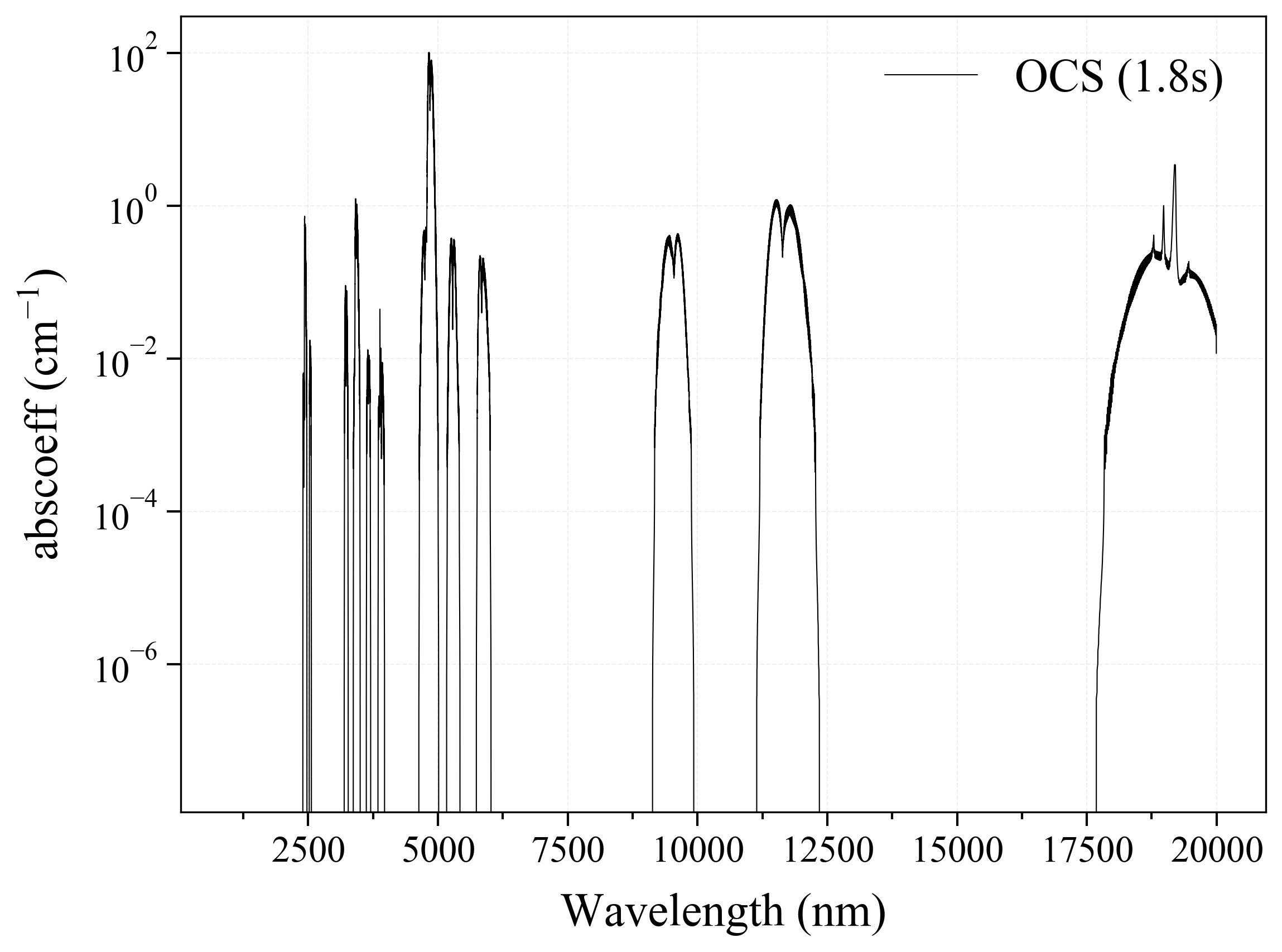## 20. H2CO¶

• 20 `'H2CO'` : Formaldehyde absorption coefficient (opacity) at 300 K

```s = calc_spectrum(wavelength_min=1000,
wavelength_max=20000,
Tgas=300,
pressure=1,
molecule='H2CO',
optimization=None,
cutoff=1e-23,
isotope='1')
s.plot('abscoeff', wunit='nm')
```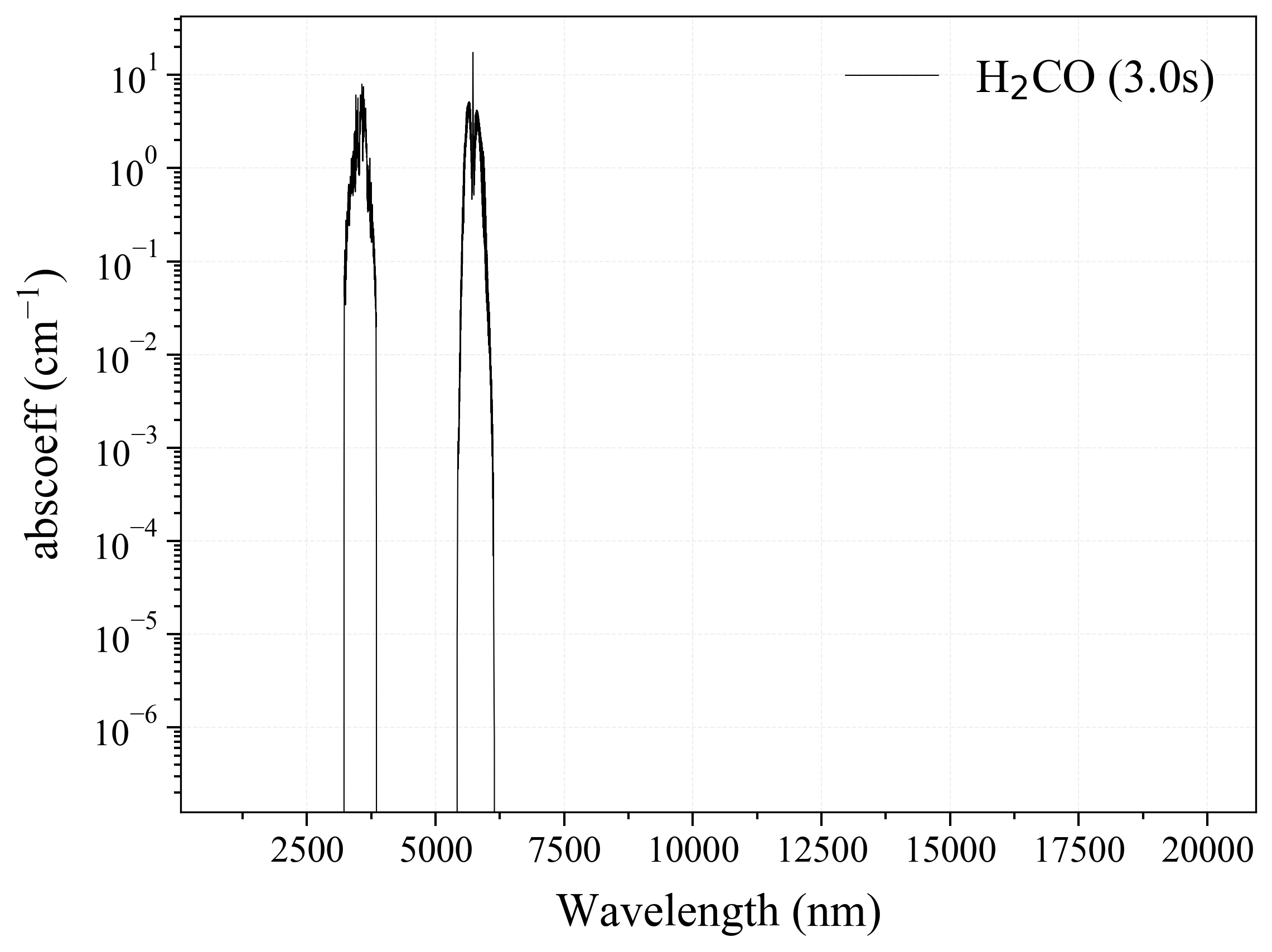## 21. HOCl¶

• 21 `'HOCl'` : Hypochlorous Acid absorption coefficient (opacity) at 300 K

```s = calc_spectrum(wavelength_min=1000,
wavelength_max=20000,
Tgas=300,
pressure=1,
molecule='HOCl',
optimization=None,
cutoff=1e-23,
isotope='1')
s.plot('abscoeff', wunit='nm')
```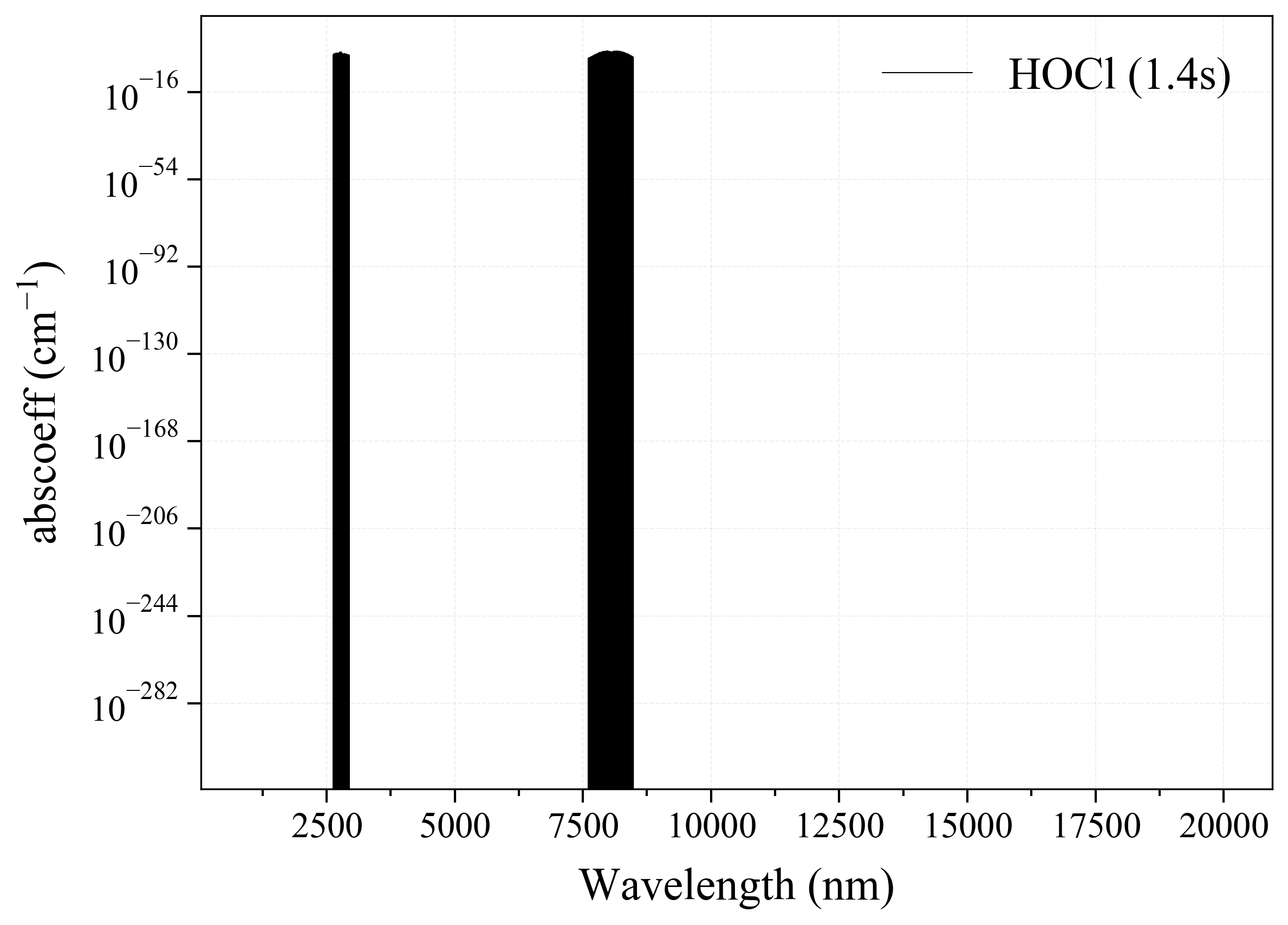## 22. N2¶

• 22 `'N2'` : Nitrogen absorption coefficient (opacity) at 300 K : no lines for `isotope='1'` (symmetric!)

## 23. HCN¶

• 23 `'HCN'`Hydrogen Cyanide absorption coefficient (opacity) at 300 Knot calculated.

## 24. CH4Cl¶

• 24 `'CH3Cl'` : Methyl Chloride absorption coefficient (opacity) at 300 K

```s = calc_spectrum(wavelength_min=1000,
wavelength_max=20000,
Tgas=300,
pressure=1,
molecule='CH3Cl',
optimization=None,
cutoff=1e-23,
isotope='1')
s.plot('abscoeff', wunit='nm')
```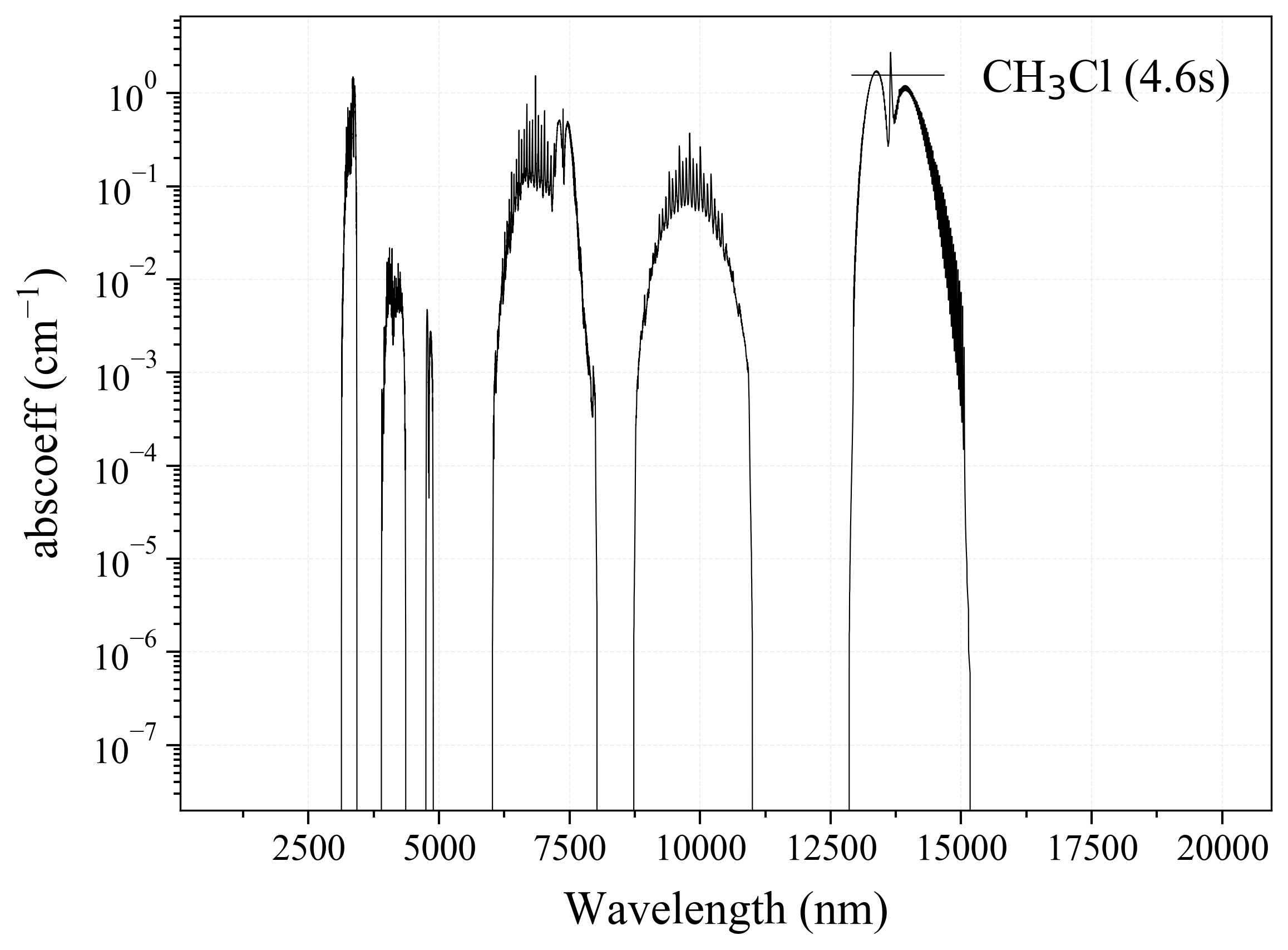## 25. H2O2¶

• 25 `'H2O2'` : Hydrogen Peroxide absorption coefficient (opacity) at 300 K

```s = calc_spectrum(wavelength_min=1000,
wavelength_max=20000,
Tgas=300,
pressure=1,
molecule='H2O2',
optimization=None,
cutoff=1e-23,
isotope='1')
s.plot('abscoeff', wunit='nm')
```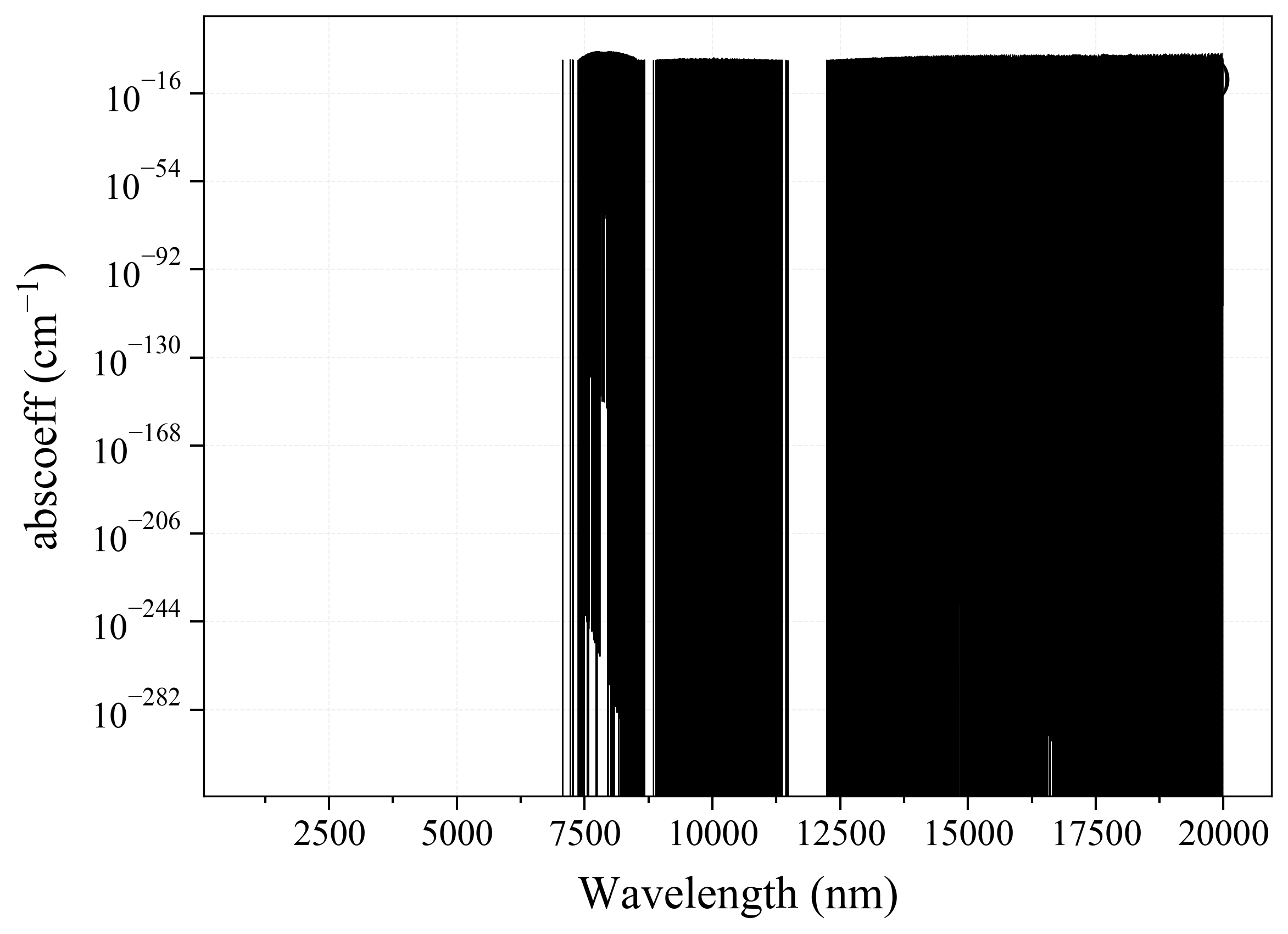## 26. C2H2¶

• 26 `'C2H2'` : Acetylene absorption coefficient (opacity) at 300 K

```s = calc_spectrum(wavelength_min=1000,
wavelength_max=20000,
Tgas=300,
pressure=1,
molecule='C2H2',
optimization=None,
cutoff=1e-23,
isotope='1')
s.plot('abscoeff', wunit='nm')
```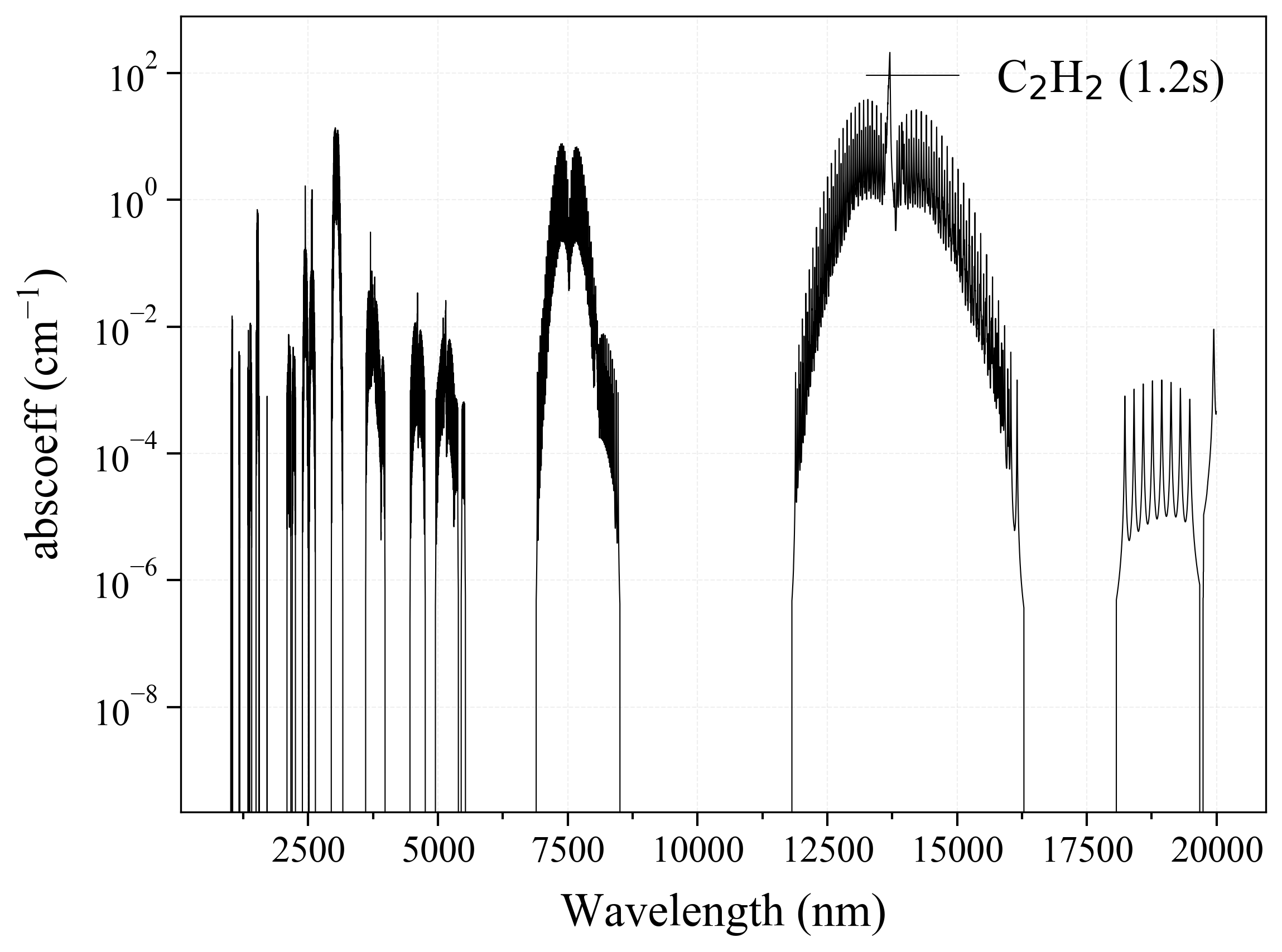## 27. C2H6¶

• 27 `'C2H6'` : Ethane absorption coefficient (opacity) at 300 K

```s = calc_spectrum(wavelength_min=1000,
wavelength_max=20000,
Tgas=300,
pressure=1,
molecule='C2H6',
optimization=None,
cutoff=1e-23,
isotope='1')
s.plot('abscoeff', wunit='nm')
```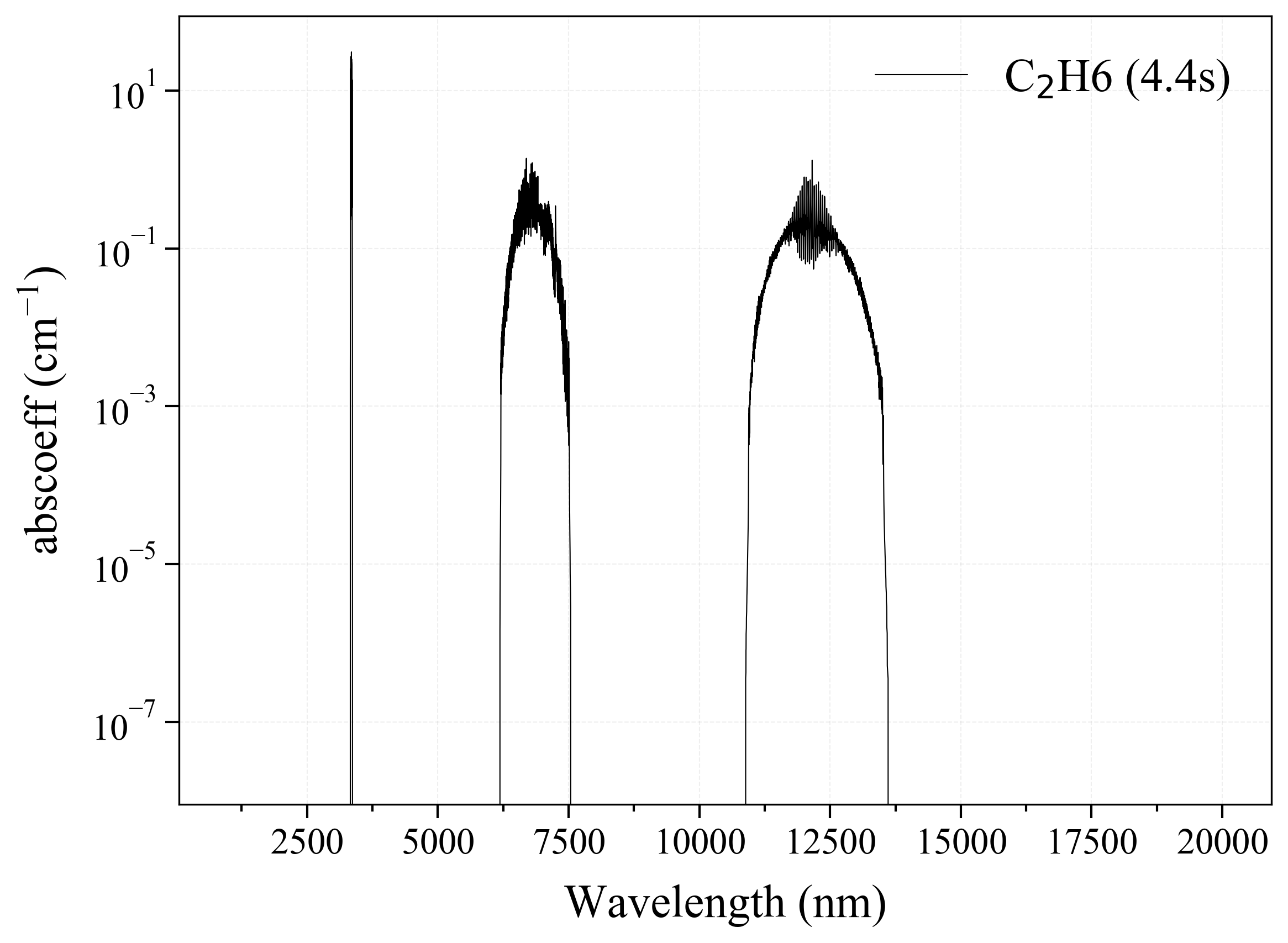## 28. PH3¶

• 28 `'PH3'` : Phosphine absorption coefficient (opacity) at 300 K

```s = calc_spectrum(wavelength_min=1000,
wavelength_max=20000,
Tgas=300,
pressure=1,
molecule='PH3',
optimization=None,
cutoff=1e-23,
isotope='1')
s.plot('abscoeff', wunit='nm')
```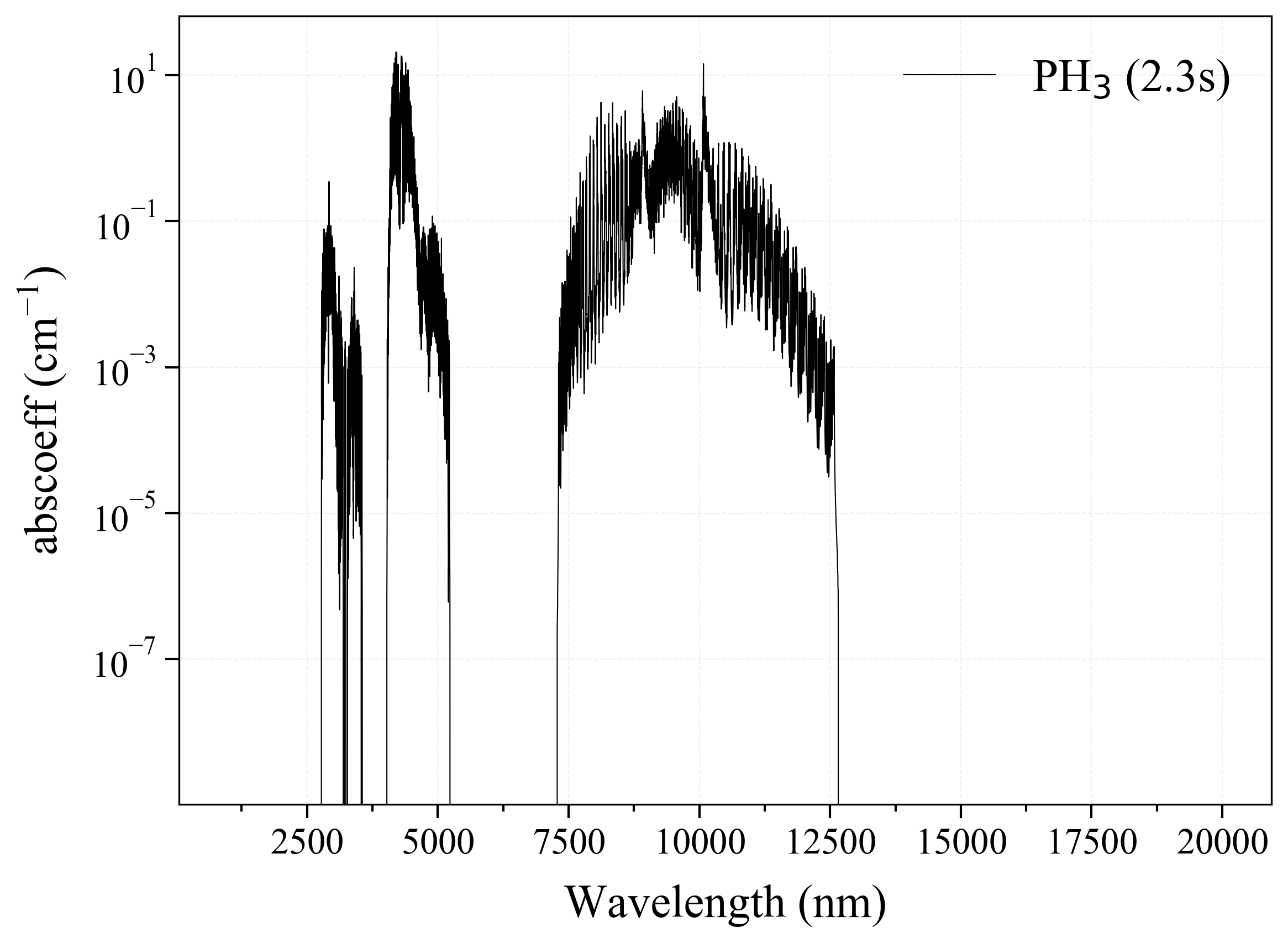## 29. COF2¶

• 29 `'COF2'` : Carbonyl Fluoride absorption coefficient (opacity) at 300 K

```s = calc_spectrum(wavelength_min=1000,
wavelength_max=20000,
Tgas=300,
pressure=1,
molecule='COF2',
optimization=None,
cutoff=1e-23,
isotope='1')
s.plot('abscoeff', wunit='nm')
```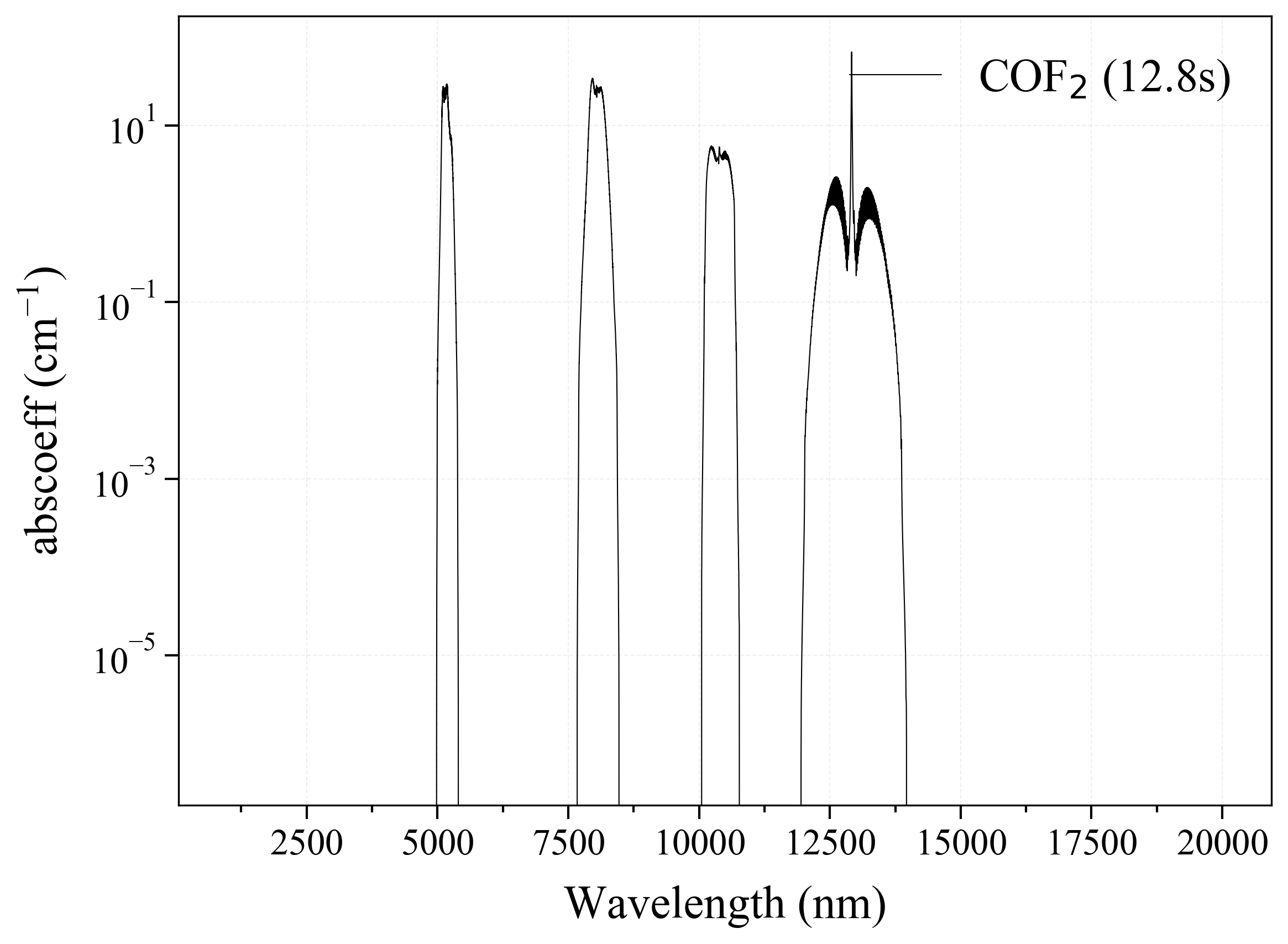## 30. SF6¶

• 30 `'SF6'`Sulfur Hexafluoride absorption coefficient (opacity) at 300 Knot calculated.

## 31. H2S¶

• 31 `'H2S'` : Hydrogen Sulfide absorption coefficient (opacity) at 300 K

```s = calc_spectrum(wavelength_min=1000,
wavelength_max=20000,
Tgas=300,
pressure=1,
molecule='H2S',
optimization=None,
cutoff=1e-23,
isotope='1')
s.plot('abscoeff', wunit='nm')
```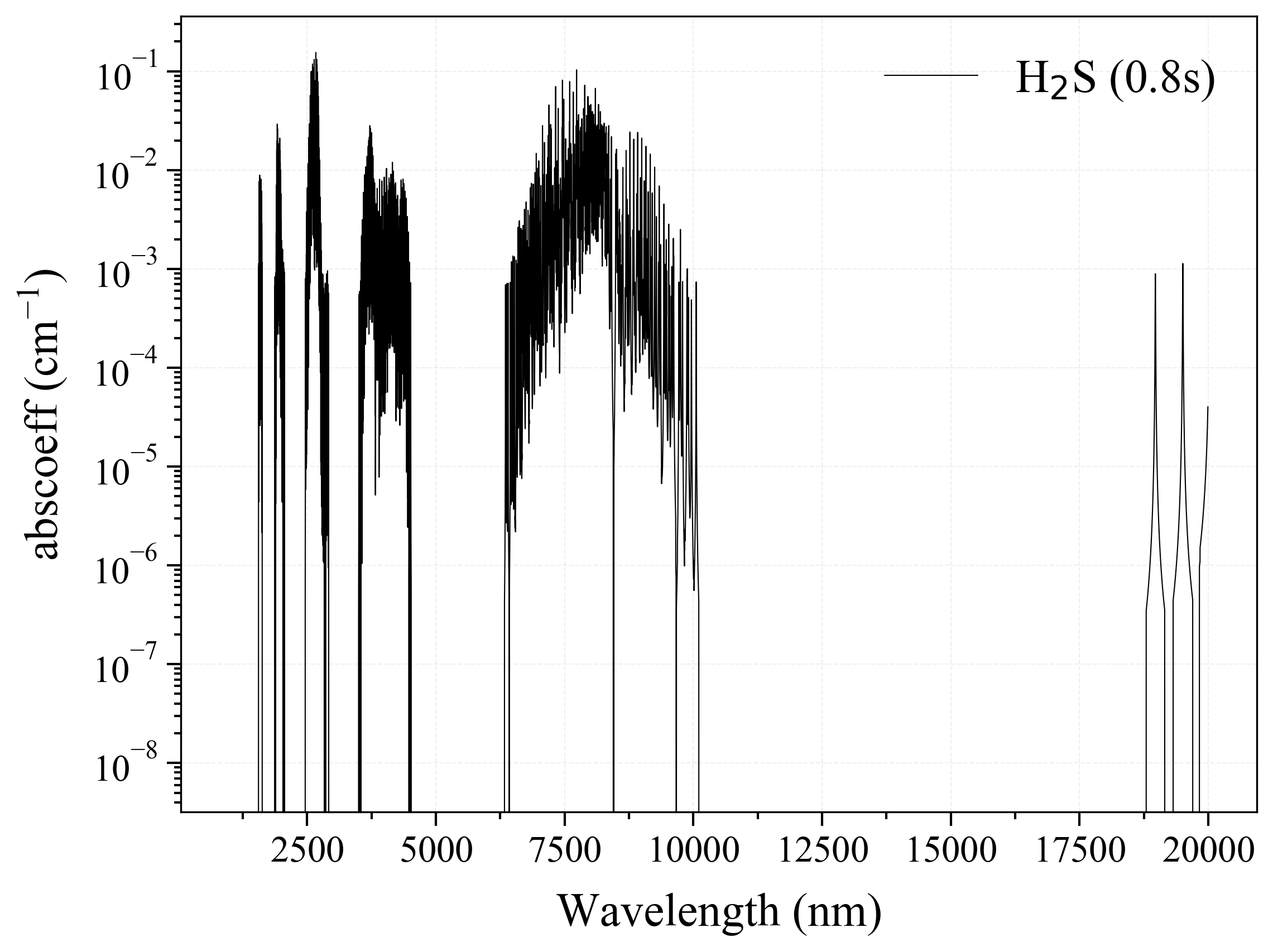## 32. HCOOH¶

• 32 `'HCOOH'` : Formic Acid absorption coefficient (opacity) at 300 K

```s = calc_spectrum(wavelength_min=1000,
wavelength_max=20000,
Tgas=300,
pressure=1,
molecule='HCOOH',
optimization=None,
cutoff=1e-23,
isotope='1')
s.plot('abscoeff', wunit='nm')
```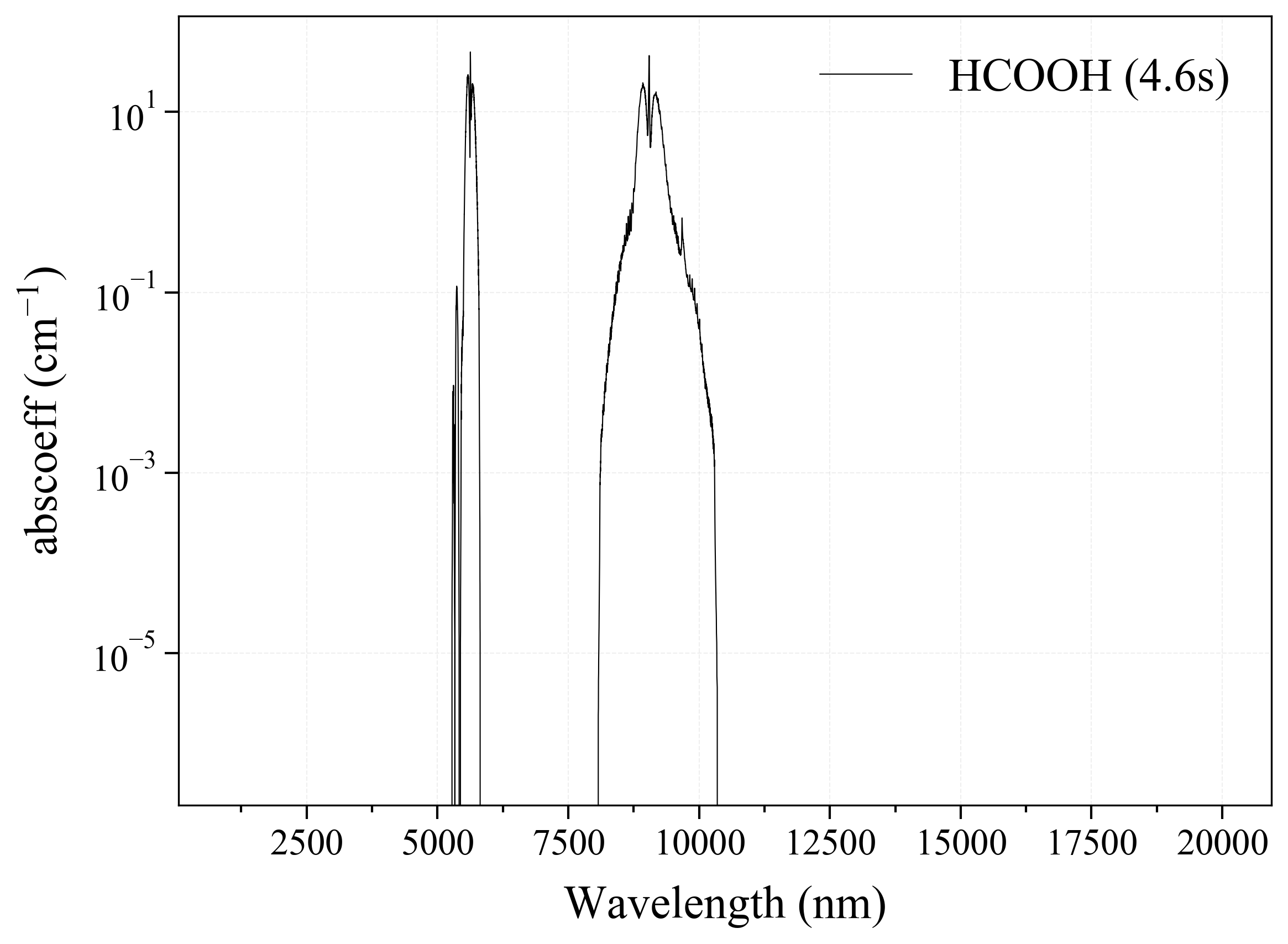## 33. HO2¶

• 33 `'HO2'` : Hydroperoxyl absorption coefficient (opacity) at 300 K

```s = calc_spectrum(wavelength_min=1000,
wavelength_max=20000,
Tgas=300,
pressure=1,
molecule='HO2',
optimization=None,
cutoff=1e-23,
isotope='1')
s.plot('abscoeff', wunit='nm')
```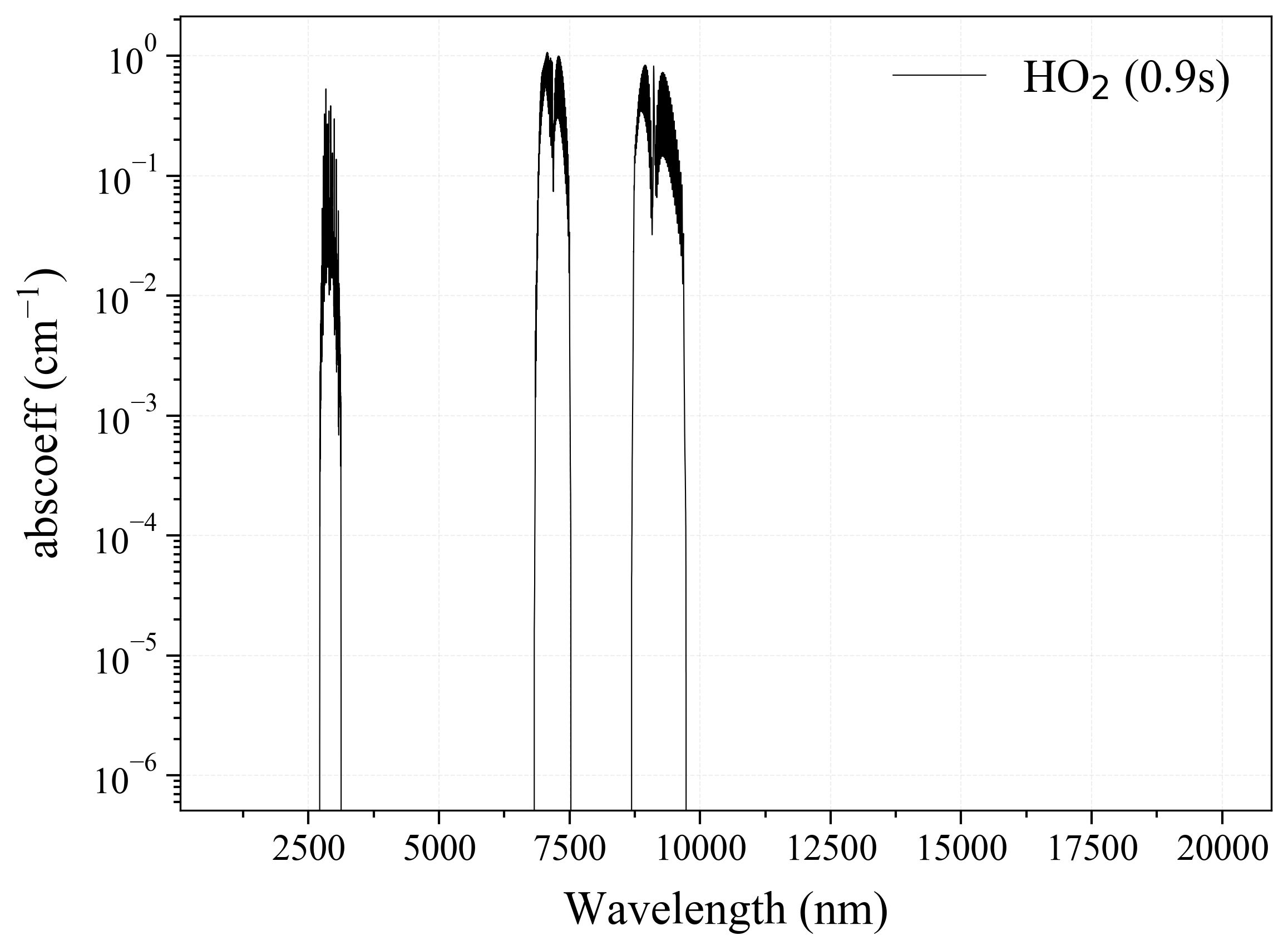34. O =====-

• 34 `'O'`Oxygen Atom absorption coefficient (opacity) at 300 Knot calculated.

## 35. ClONO2¶

• 35 `'ClONO2'`Chlorine Nitrate absorption coefficient (opacity) at 300 Knot calculated.

## 36. NO+¶

• 36 `'NO+'` : Nitric Oxide Cation absorption coefficient (opacity) at 300 K

```s = calc_spectrum(wavelength_min=1000,
wavelength_max=20000,
Tgas=300,
pressure=1,
molecule='NO+',
optimization=None,
cutoff=1e-23,
isotope='1')
s.plot('abscoeff', wunit='nm')
```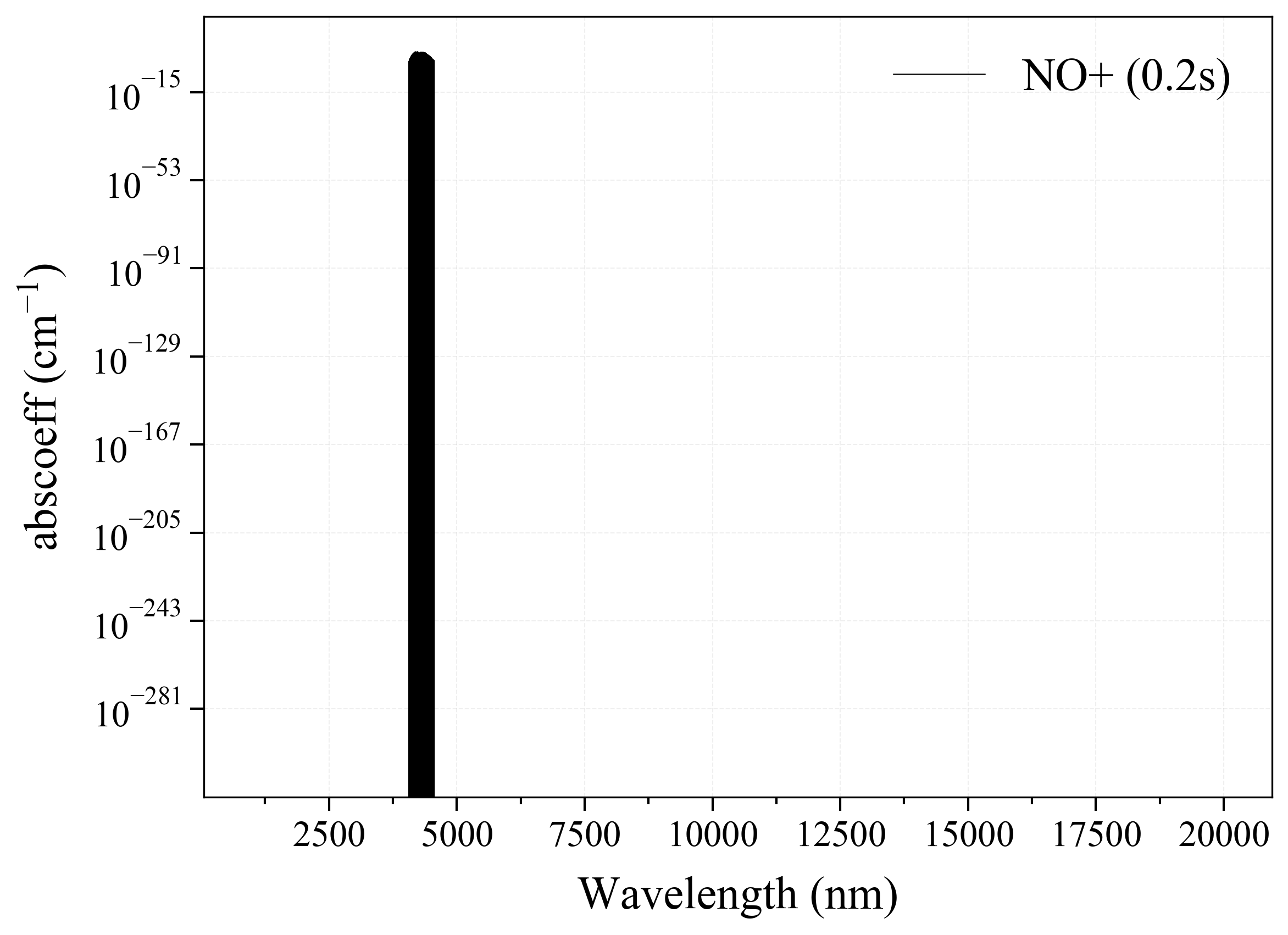## 37. HOBr¶

• 37 `'HOBr'`Hypobromous Acid absorption coefficient (opacity) at 300 Knot calculated.

## 38. C2H4¶

• 38 `'C2H4'`Ethylene absorption coefficient (opacity) at 300 Knot calculated.

## 39. CH3OH¶

• 39 `'CH3OH'`Methanol absorption coefficient (opacity) at 300 Knot calculated.

## 40. CH3Br¶

• 40 `'CH3Br'`Methyl Bromide absorption coefficient (opacity) at 300 Knot calculated.

## 41. CH3CN¶

• 41 `'CH3CN'`Acetonitrile absorption coefficient (opacity) at 300 Knot calculated.

## 42. CF4¶

• 42 `'CF4'`CFC-14 absorption coefficient (opacity) at 300 Knot calculated.

## 43. C4H2¶

• 43 `'C4H2'`Diacetylene absorption coefficient (opacity) at 300 Knot calculated.

## 44. HC3N¶

• 44 `'HC3N'`Cyanoacetylene absorption coefficient (opacity) at 300 Knot calculated.

## 45. H2¶

• 45 `'H2'`Hydrogen absorption coefficient (opacity) at 300 Knot calculated.

## 46. CS¶

• 46 `'CS'`Carbon Monosulfide absorption coefficient (opacity) at 300 Knot calculated.

## 47. SO3¶

• 47 `'SO3'`Sulfur trioxide absorption coefficient (opacity) at 300 Knot calculated.

## 48. C2N2¶

• 48 `'C2N2'`Cyanogen absorption coefficient (opacity) at 300 Knot calculated.

## 49. COCl2¶

• 49 `'COCl2'`Phosgene absorption coefficient (opacity) at 300 Knot calculated.